# Making smoothed scatterplots in python

The other day I made a blog post on my notes on making scatterplots in matplotlib. One big chunk of why you want to make scatterplots though is if you are interested in a predictive relationship. Typically you want to look at the conditional value of the Y variable based on the X variable. Here are some example exploratory data analysis plots to accomplish that task in python.

I have posted the code to follow along on github here, in particular smooth.py has the functions of interest, and below I have various examples (that are saved in the Examples_Conditional.py file).

# Data Prep

First to get started, I am importing my libraries and loading up some of the data from my dissertation on crime in DC at street units. My functions are in the smooth set of code. Also I change the default matplotlib theme using smooth.change_theme(). Only difference from my prior posts is I don’t have gridlines by default here (they can be a bit busy).

#################################
import pandas as pd
import numpy as np
import matplotlib.pyplot as plt
import seaborn as sns
import statsmodels.api as sm
import os
import sys

mydir = r'D:\Dropbox\Dropbox\PublicCode_Git\Blog_Code\Python\Smooth'
data_loc = r'https://dl.dropbox.com/s/79ma3ldoup1bkw6/DC_CrimeData.csv?dl=0'
os.chdir(mydir)

#My functions
sys.path.append(mydir)
import smooth
smooth.change_theme()

#Dissertation dataset, can read from dropbox
#################################

# Binned Conditional Plots

The first set of examples, I bin the data and estimate the conditional means and standard deviations. So here in this example I estimate E[Y | X = 0], E[Y | X = 1], etc, where Y is the total number of part 1 crimes and x is the total number of alcohol licenses on the street unit (e.g. bars, liquor stores, or conv. stores that sell beer).

The function name is mean_spike, and you pass in at a minimum the dataframe, x variable, and y variable. I by default plot the spikes as +/- 2 standard deviations, but you can set it via the mult argument.

####################
#Example binning and making mean/std dev spike plots

smooth.mean_spike(DC_crime,'TotalLic','TotalCrime')

mean_lic = smooth.mean_spike(DC_crime,'TotalLic','TotalCrime',
plot=False,ret_data=True)
####################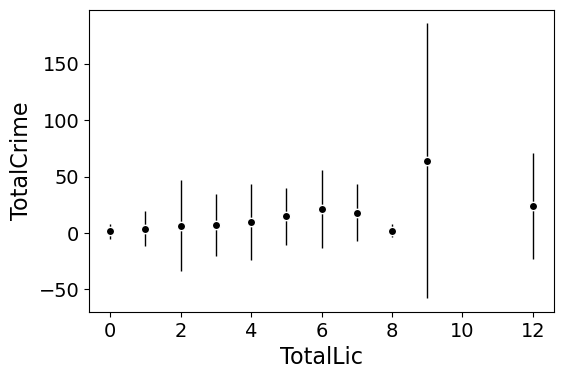This example works out because licenses are just whole numbers, so it can be binned. You can pass in any X variable that can be binned in the end. So you could pass in a string for the X variable. If you don’t like the resulting format of the plot though, you can just pass plot=False,ret_data=True for arguments, and you get the aggregated data that I use to build the plots in the end.

mean_lic = smooth.mean_spike(DC_crime,'TotalLic','TotalCrime',
plot=False,ret_data=True)

Another example I am frequently interested in is proportions and confidence intervals. Here it uses exact binomial confidence intervals at the 99% confidence level. Here I clip the burglary data to 0/1 values and then estimate proportions.

####################
#Example with proportion confidence interval spike plots

DC_crime['BurgClip'] = DC_crime['OffN3'].clip(0,1)
smooth.prop_spike(DC_crime,'TotalLic','BurgClip')

####################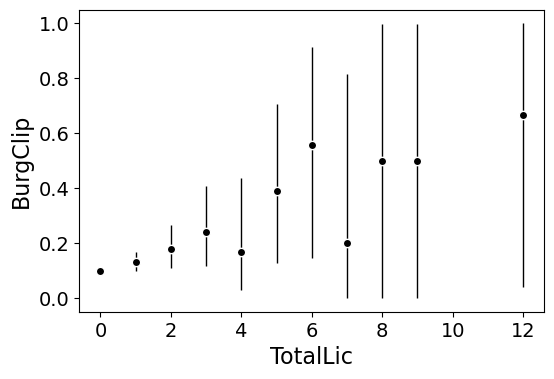A few things to note about this is I clip out bins with only 1 observation in them for both of these plots. I also do not have an argument to save the plot. This is because I typically only use these for exploratory data analysis, it is pretty rare I use these plots in a final presentation or paper.

I will need to update these in the future to jitter the data slightly to be able to superimpose the original data observations. The next plots are a bit easier to show that though.

# Restricted Cubic Spline Plots

Binning like I did prior works out well when you have only a few bins of data. If you have continuous inputs though it is tougher. In that case, typically what I want to do is estimate a functional relationship in a regression equation, e.g. Y ~ f(x), where f(x) is pretty flexible to identify potential non-linear relationships.

Many analysts are taught the loess linear smoother for this. But I do not like loess very much, it is often both locally too wiggly and globally too smooth in my experience, and the weighting function has no really good default.

Another popular choice is to use generalized additive model smoothers. My experience with these (in R) is better than loess, but they IMO tend to be too aggressive, and identify overly complicated functions by default.

My favorite approach to this is actually then from Frank Harrell’s regression modeling strategies. Just pick a regular set of restricted cubic splines along your data. It is arbitrary where to set the knot locations for the splines, but my experience is they are very robust (so chaning the knot locations only tends to change the estimated function form by a tiny bit).

I have class notes on restricted cubic splines I think are a nice introduction. First, I am going to make the same dataset from my class notes, the US violent crime rate from 85 through 2010.

years = pd.Series(list(range(26)))
vcr = [1881.3,
1995.2,
2036.1,
2217.6,
2299.9,
2383.6,
2318.2,
2163.7,
2089.8,
1860.9,
1557.8,
1344.2,
1268.4,
1167.4,
1062.6,
945.2,
927.5,
789.6,
734.1,
687.4,
673.1,
637.9,
613.8,
580.3,
551.8,
593.1]

yr_df = pd.DataFrame(zip(years,years+1985,vcr), columns=['y1','years','vcr'])

I have a function that allows you to append the spline basis to a dataframe. If you don’t pass in a data argument, in returns a dataframe of the basis functions.

#Can append rcs basis to dataframe
kn = [3.0,7.0,12.0,21.0]
smooth.rcs(years,knots=kn,stub='S',data=yr_df)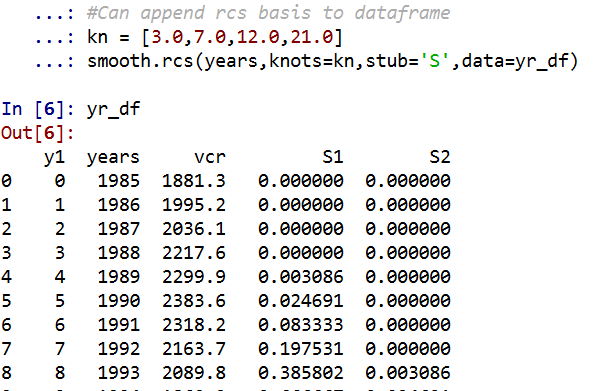I also have in the code set Harrell’s suggested knot locations for the data. This ranges from 3 to 7 knots (it will through an error if you pass a number not in that range). This here suggests the locations [1.25, 8.75, 16.25, 23.75].

#If you want to use Harrell's rules to suggest knot locations
smooth.sug_knots(years,4)

Note if you have integer data here these rules don’t work out so well (can have redundant suggested knot locations). So Harell’s defaults don’t work with my alcohol license data. But it is one of the reasons I like these though, I just pick regular locations along the X data and they tend to work well. So here is a regression plot passing in those knot locations kn = [3.0,7.0,12.0,21.0] I defined a few paragraphs ago, and the plot does a few vertical guides to show the knot locations.

#RCS plot
smooth.plot_rcs(yr_df,'y1','vcr',knots=kn)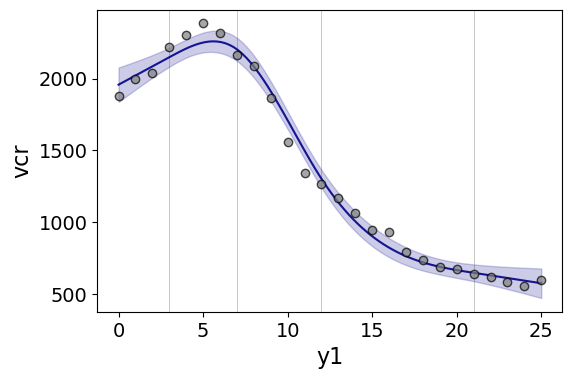Note that the error bands in the plot are confidence intervals around the mean, not prediction intervals. One of the nice things though about this under the hood, I used statsmodels glm interface, so if you want you can change the underlying link function to Poisson (I am going back to my DC crime data here), you just pass it in the fam argument:

#Can pass in a family argument for logit/Poisson models
smooth.plot_rcs(DC_crime,'TotalLic','TotalCrime', knots=[3,7,10,15],
fam=sm.families.Poisson(), marker_size=12)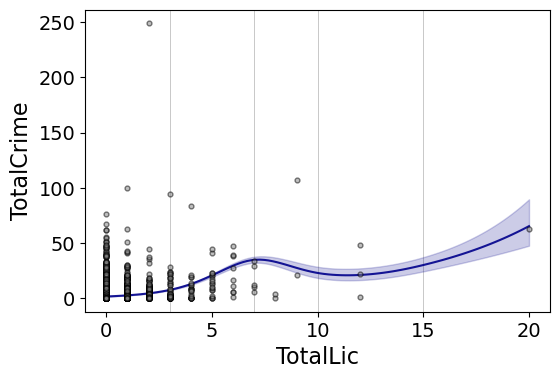This is a really great example for the utility of splines. I will show later, but a linear Poisson model for the alcohol license effect extrapolates very poorly and ends up being explosive. Here though the larger values the conditional effect fits right into the observed data. (And I swear I did not fiddle with the knot locations, there are just what I picked out offhand to spread them out on the X axis.)

And if you want to do a logistic regression:

smooth.plot_rcs(DC_crime,'TotalLic','BurgClip', knots=[3,7,10,15],
fam=sm.families.Binomial(),marker_alpha=0)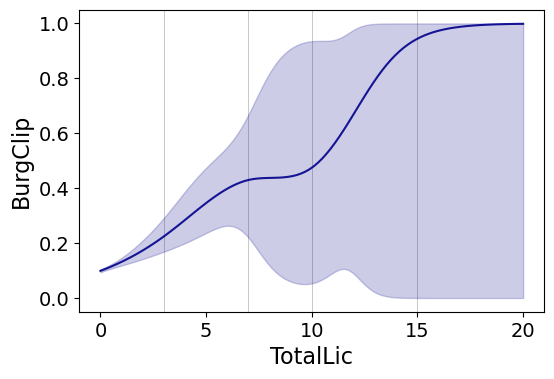I’m not sure how to do this in a way you can get prediction intervals (I know how to do it for Gaussian models, but not for the other glm families, prediction intervals probably don’t make sense for binomial data anyway). But one thing I could expand on in the future is to do quantile regression instead of glm models.

# Smooth Plots by Group

Sometimes you want to do the smoothed regression plots with interactions per groups. I have two helper functions to do this. One is group_rcs_plot. Here I use the good old iris data to illustrate, which I will explain why in a second.

#Superimposing rcs on the same plot
smooth.group_rcs_plot(iris,'sepal_length','sepal_width',
'species',colors=None,num_knots=3)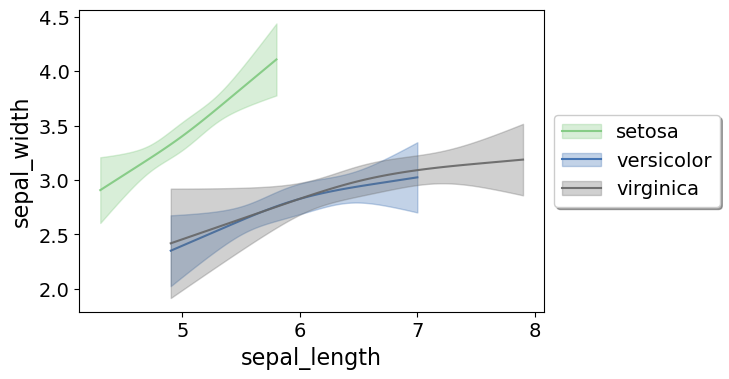If you pass in the num_knots argument, the knot locations are different for each subgroup of data (which I like as a default). If you pass in the knots argument and the locations, they are the same though for each subgroup.

Note that the way I estimate the models here I estimate three different models on the subsetted data frame, I do not estimate a stacked model with group interactions. So the error bands will be a bit wider than estimating the stacked model.

Sometimes superimposing many different groups is tough to visualize. So then a good option is to make a set of small multiple plots. To help with this, I’ve made a function loc_error, to pipe into seaborn’s small multiple set up:

#Small multiple example
g = sns.FacetGrid(iris, col='species',col_wrap=2)
g.map_dataframe(smooth.loc_error, x='sepal_length', y='sepal_width', num_knots=3)
g.set_axis_labels("Sepal Length", "Sepal Width")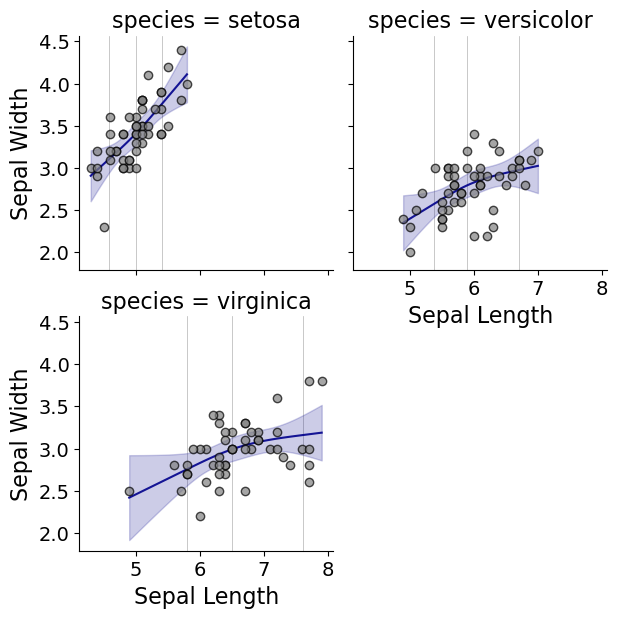And here you can see that the not locations are different for each subset, and this plot by default includes the original observations.

# Using the Formula Interface for Plots

Finally, I’ve been experimenting a bit with using the input in a formula interface, more similar to the way ggplot in R allows you to do this. So this is a new function, plot_form, and here is an example Poisson linear model:

smooth.plot_form(data=DC_crime,x='TotalLic',y='TotalCrime',
form='TotalCrime ~ TotalLic',
fam=sm.families.Poisson(), marker_size=12)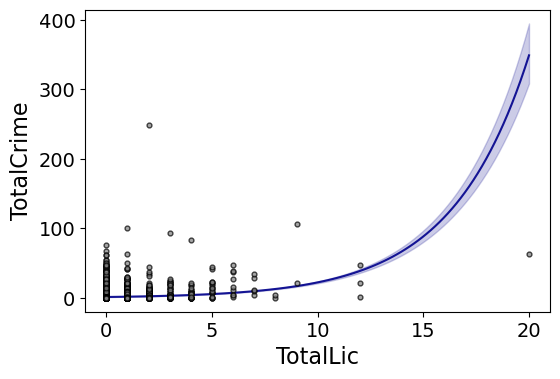You can see the explosive effect I talked about, which is common for Poisson/negative binomial models.

Here with the formula interface you can do other things, such as a polynomial regression:

#Can do polynomial terms
smooth.plot_form(data=DC_crime,x='TotalLic',y='TotalCrime',
form='TotalCrime ~ TotalLic + TotalLic**2 + TotalLic**3',
fam=sm.families.Poisson(), marker_size=12)

Which here ends up being almost indistinguishable from the linear terms. You can do other smoothers that are available in the patsy library as well, here are bsplines:

#Can do other smoothers
smooth.plot_form(data=DC_crime,x='TotalLic',y='TotalCrime',
form='TotalCrime ~ bs(TotalLic,df=4,degree=3)',
fam=sm.families.Poisson(), marker_size=12)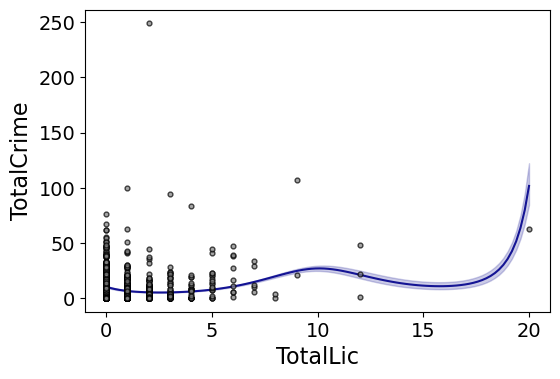I don’t really have a good reason to prefer restricted cubic splines to bsplines, I am just more familiar with restricted cubic splines (and this plot does not illustrate the knot locations that were by default chosen, although you could pass in knot locations to the bs function).

You can also do other transformations of the x variable. So here if you take the square root of the total number of licenses helps with the explosive effect somewhat:

#Can do transforms of the X variable
smooth.plot_form(data=DC_crime,x='TotalLic',y='TotalCrime',
form='TotalCrime ~ np.sqrt(TotalLic)',
fam=sm.families.Poisson(), marker_size=12)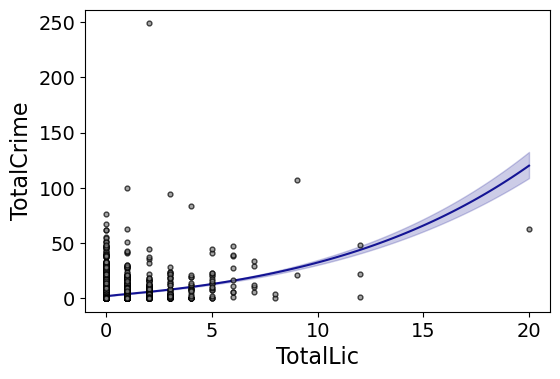In the prior blog post about explosive Poisson models I also showed a broken stick type model if you wanted to log the x variable but it has zero values.

#Can do multiple transforms of the X variable
smooth.plot_form(data=DC_crime,x='TotalLic',y='TotalCrime',
form='TotalCrime ~ np.log(TotalLic.clip(1)) + I(TotalLic==0)',
fam=sm.families.Poisson(), marker_size=12)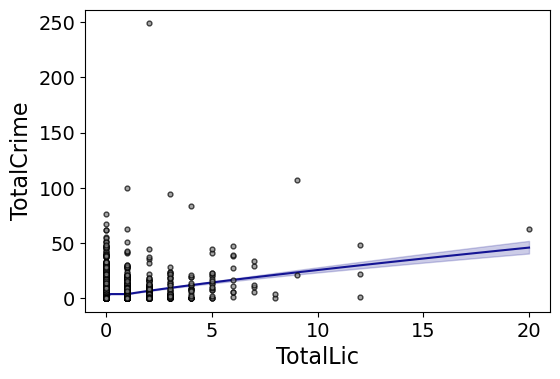Technically this “works” if you transform the Y variable as well, but the resulting plot is misleading, and the prediction interval is for the transformed variable. E.g. if you pass a formula 'np.log(TotalCrime+1) ~ TotalLic', you would need to exponentiate the the predictions and subtract 1 to get back to the original scale (and then the line won’t be the mean anymore, but the confidence intervals are OK).

I will need to see if I can figure out patsy and sympy to be able to do the inverse transformation to even do that. That type of transform to the y variable directly probably only makes sense for linear models, and then I would also maybe need to do a Duan type smearing estimate to get the mean effect right.

# Making aoristic density maps in R

I saw Jerry the other day made/updated an R package to do aoristic analysis. A nice part of this is that it returns the weights breakdown for individual cases, which you can then make maps of. My goto hot spot map for data visualization, kernel density maps, are a bit tough to work with weighted data though in R (tough is maybe not the right word, to use ggplot it takes a bit of work leveraging other packages). So here are some notes on that.

I have provided the data/code here. It is burglaries in Dallas, specifically I filter out just for business burglaries.

# R Code Snippet

First, for my front end I load the libraries I will be using, and change the working directory to where my data is located.

############################
library(aoristic) #aoristic analysis
library(rgdal)    #importing spatial data
library(spatstat) #weighted kde
library(raster)   #manipulate raster object
library(ggplot2)  #for contour graphs
library(sf)       #easier to plot sf objects

my_dir <- "D:\\Dropbox\\Dropbox\\Documents\\BLOG\\aoristic_maps_R\\data_analysis"
setwd(my_dir)
############################

Next I just have one user defined function, this takes an input polygon (the polygon that defines the borders of Dallas here), and returns a raster grid covering the bounding box. It also have an extra data field, to say whether the grid cell is inside/outside of the boundary. (This is mostly convenient when creating an RTM style dataset to make all the features conform to the same grid cells.)

###########################
#Data Manipulation Functions

#B is border, g is size of grid cell on one side
BaseRaster <- function(b,g){
base_raster <- raster(ext = extent(b), res=g)
projection(base_raster) <- crs(b)
mask_raster <- rasterize(b, base_raster, getCover=TRUE) #percentage of cover, 0 is outside
}
###########################

The next part I grab the datasets I will be using, a boundary file for Dallas (in which I chopped off the Lochs, so will not be doing an analysis of boat house burglaries today), and then the crime data. R I believe you always have to convert date-times when reading from a CSV (it never smartly infers that a column is date/time). And then I do some other data fiddling – Jerry has a nice function to check and make sure the date/times are all in order, and then I get rid of points outside of Dallas using the sp over function. Finally the dataset is for both residential/commercial, but I just look at the commercial burglaries here.

###########################
#Get the datasets

#Geo data
base_Dallas <- BaseRaster(b=boundary,g=200)
base_df <- as.data.frame(base_Dallas,long=TRUE,xy=TRUE)

#Crime Data
#prepping time fields
crime_dat$Beg <- as.POSIXct(crime_dat$StartingDateTime, format="%m/%d/%Y %H:%M:%OS")
crime_dat$End <- as.POSIXct(crime_dat$EndingDateTime, format="%m/%d/%Y %H:%M:%OS")

#cleaning up data
aor_check <- aoristic.datacheck(crime_dat, 'XCoordinate', 'YCoordinate', 'Beg', 'End')
coordinates(crime_dat) <- crime_dat[,c('XCoordinate', 'YCoordinate')]
crs(crime_dat) <- crs(boundary)
over_check <- over(crime_dat, boundary)
keep_rows <- (aor_check$aoristic_datacheck == 0) & (!is.na(over_check$city))
crime_dat_clean <- crime_dat[keep_rows,]

#only look at business burgs to make it go abit faster
busi_burgs <- crime_dat_clean[ crime_dat_clean$UCROffense == 'BURGLARY-BUSINESS', ] ########################### The next part preps the aoristic weights. First, the aoristic.df function is from Jerry’s aoristic package. It returns the weights broken down by 168 hours per day of the week. Here I then just collapse across the weekdays into the same hour, which to do that is simple, just add up the weights. After that it is some more geographic data munging using the spatstat package to do the heavy lifting for the weighted kernel density estimate, and then stuffing the result back into another data frame. My bandwidth here, 3000 feet, is a bit large but makes nicer looking maps. If you do this smaller you will have a more bumpy and localized hot spots in the kernel density estimate. ########################### #aoristic weights #This takes like a minute res_weights <- aoristic.df(busi_burgs@data, 'XCoordinate', 'YCoordinate', 'Beg', 'End') #Binning into same hourly bins for (i in 1:24){ cols <- (0:6*24)+i+5 lab <- paste0("Hour",i) res_weights[,c(lab)] <- rowSums(res_weights[,cols]) } #Prepping the spatstat junk I need peval <- rasterToPoints(base_Dallas)[,1:2] spWin <- as.owin(as.data.frame(peval)) sp_ppp <- as.ppp(res_weights[,c('x_lon','y_lat')],W=spWin) #spp point pattern object #Creating a dataframe with all of the weighted KDE Hour_Labs <- paste0("Hour",1:24) for (h in Hour_Labs){ sp_den <- density.ppp(sp_ppp,weights=res_weights[,c(h)], sigma=3000, edge=FALSE,warnings=FALSE) sp_dat <- as.data.frame(sp_den) kd_raster <- rasterFromXYZ(sp_dat,res=res(base_Dallas),crs=crs(base_Dallas)) base_df[,c(h)] <- as.data.frame(kd_raster,long=TRUE)$value
}
###########################

If you are following along, you may be wondering why all the hassle? It is partly because I want to use ggplot to make maps, but for its geom_contour it does not except weights, so I need to do the data manipulation myself to supply ggplot the weighted data in the proper format.

First I turn my Dallas boundary into a simple feature sf object, then I create my filled contour graph, supplying the regular grid X/Y and the Z values for the first Hour of the day (so between midnight and 1 am).

###########################
#now making contour graphs

dallas_sf <- st_as_sf(boundary)

#A plot for one hour of the day
hour1 <- ggplot() +
geom_contour_filled(data=base_df, aes(x, y, z = Hour1), bins=9) +
geom_sf(data=dallas_sf, fill=NA, color='black') +
scale_fill_brewer(palette="Greens") +
ggtitle('       Hour [0-1)') +
theme_void() + theme(legend.position = "none")
hour1

png('Hour1.png', height=5, width=5, units="in", res=1000, type="cairo")
hour1
dev.off()
###########################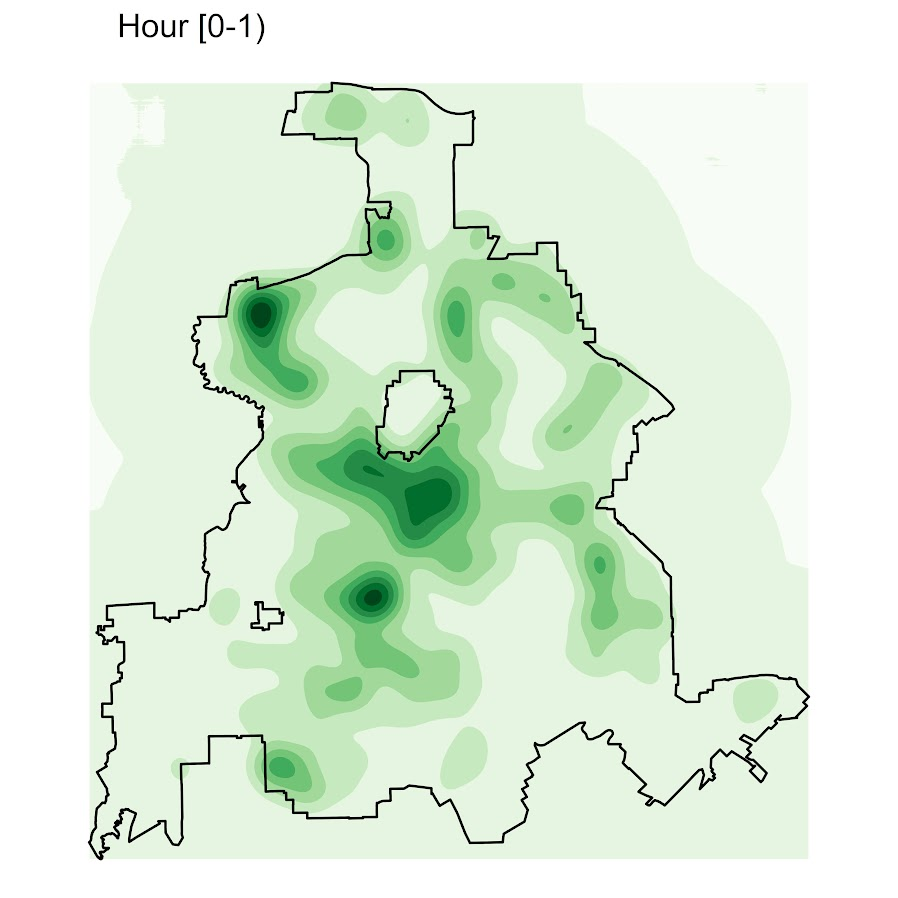Nice right! I have in the code my attempt to make a super snazzy small multiple plot, but that was not working out so well for me. But you can then go ahead and make up other slices if you want. Here is an example of taking an extended lunchtime time period.

###########################
#Plot for the afternoon time period
base_df$Afternoon <- rowSums(base_df[,paste0("Hour",10:17)]) afternoon <- ggplot() + geom_contour_filled(data=base_df, aes(x, y, z = Afternoon), bins=9) + geom_sf(data=dallas_sf, fill=NA, color='black') + scale_fill_brewer(palette="Greens") + ggtitle(' Hour [9:00-17:00)') + theme_void() + theme(legend.position = "none") afternoon ###########################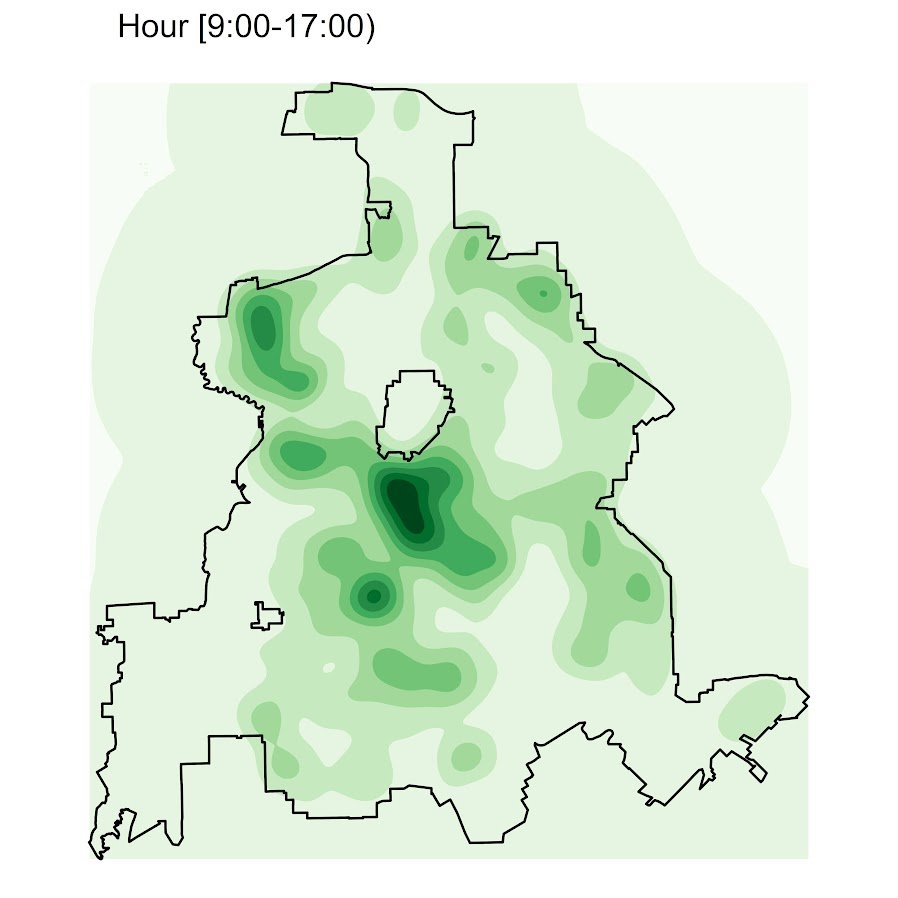So you can see that the patterns only slightly changed compared to the midnight prior graph. Note that these plots will have different breaks, but you could set them to be equal by simply specifying a breaks argument in the geom_contour_filled call. I will leave it up so someone who is more adept at R code than me to make a cool animated viz over time from this. But that is a way to mash up the temporal weights in a map. # Deleted Twitter Account I’ve decided to delete Twitter. It is for multiple reasons in the end. Reason 1, I was definitely addicted to it. Checked it quite often during the daytime. Deleting off of my phone (and ditto for email) was a good first step, but I still checked it quite a bit when I was on my personal computer. Reason 2 — there is a XKCD comic about staying up arguing with people on the internet. I was constantly tempted to do this on Twitter. It is never really worth it. Many of the examples that come to mind I did this — had a comment stream with Pete Kraska the other day about grant funding, and in the past Travis Pratt over pre-prints — Pete/Travis had an ounce of truth in their initial statements, but made sweeping generalizations that don’t describe the majority of people (which included me, hence my urge to respond). While they likely did not intend to say something directly about me, they did so in making general stereotyping comments. I respect each as scholars, but they just have ill-informed opinions in those cases. You would think criminologists would be less likely to attribute the malice of a few to widespread groups of individuals, but so it goes. No doubt I have bad/wrong opinions all the time as well. Reason 3, a former colleague the other day was upset I liked a tweet that was a critique of their work. This is just one example, but there are a million different things people could take offense to. I am not interested in even the potential of saying or doing something that would result in a sandbag onslaught I’ve seen several times on Twitter. I of course do not intentionally mean to hurt peoples feelings, but I do not feel like defending minor stuff like that either. Worrying about things like that is just not good for my mental health. There are of course good things I will be missing out on. I initially joined Twitter to keep up on the news. Between Google Scholar and CrimPapers I can keep up on academic work. (Actual news I should definately not be getting my info from tweets!) But the biggest benefit in the end was there are several internet friends I only met on Twitter and would not have had the opportunity to meet without Twitter. And of course it was nice to tweet a blog post and get a dozen likes (or say something snarky and get 30). So my work will have less exposure than before, but honestly it was not much to begin with. My last post had more likes (around a dozen) than referrals from Twitter (around half that!) Not like tweeting my blog posts resulted in 1000’s of views, more like a few dozen extra most of the time (and a few hundred extra in the best of times). So I will just continue to write blog posts, and they will have a few less views than before. I wish my blog had bigger reach but it is really just my place for creative output. I encourage folks to always reach out and send me an email to keep in touch if you are one of my former Twitter friends. Academia can be a lonely place in normal times, and with isolating in the pandemic I can’t even imagine what it would be like without my family. I don’t think my time spent on Twitter was good for my personal well being though in the end, even though it did definitely help me be part of a larger community of colleagues and friends. # RTM Deep Learning Style In my quest to better understand deep learning, I have attempted to replicate some basic models I am familiar with in criminology, just typical OLS and the more complicated group based trajectory models. Another example I will illustrate is doing a variant of Risk Terrain Modeling. The typical way RTM is done is: Data Prep Part: 1. create a set of independent variables for crime generators (e.g. bars, subway stops, liquor stores, etc.) that are either the distance to the nearest or the kernel density estimate 2. Turn these continuous estimates into dummy variables, e.g. if within 100 meters = 1, else = 0. For kernel density they typically z-score and if a z-score > 2 the dummy variable equals 1. 3. Do 2 for varying distance/bandwidth selections, e.g. 100 meters, 200 meters, etc. So you end up with a collection of distance variables, e.g. Bars_100, Bars_200, Bars_400, etc. Modeling Part 1. Fit a Lasso regression predicting your crime outcome constraining all of the variables to be positive. (So RTM will never say a crime generator has a negative effect.) 2. For the variables that passed this Lasso stage, then do a variable selection routine. So instead of the final model having Bars_100 and Bars_400, it will only choose one of those variables. For the modeling part, we can replicate various parts of these in a deep learning environment. For the constrain the coefficients to be positive, when you see folks refer to a “RelU” or the rectified linear unit function, all this means is that the coefficients are constrained to be positive. For the variable selection part, I needed to hack my own – it ends up being a combo of a custom dropout scheme and then pruning in deep learning lingo. Although RTM is typically done on raster grid cells for the spatial unit of analysis, this is not a requirement. You can do all these steps on vector (e.g. street segments) or other areal spatial units of analysis. Here I illustrate using street units (intersections and street segments) from DC. The crime generator data I take from my dissertation (and I have a few pubs in Crime & Delinquency based on that work). The crime data I illustrate using 2011 violent Part 1 UCR (homicide, agg assault, robbery, no rape in the public data). The crime dataset is over time, and I describe in an analysis (with Billy Zakrzewski) on examining pre/post crime around DC medical marijuana dispensaries. The data and code to replicate can be downloaded here. It is python, and for the deep learning model I used pytorch. # RTM Example in Python So I will walk through briefly my second script, 01_DeepLearningRTM.py. The first script, 00_DataPrep.py, does the data prep, so this data file already has the crime generator variables prepared in the manner RTM typically creates them. (The rtm_dl_funcs.py has the functions to do the feature extraction and create the deep learning model, to do distance/density in sci-kit is very slick, only a few lines of code.) So first I just define the libraries I will be using, and import my custom rtm functions I created. ###################################################### import numpy as np import pandas as pd import torch device = torch.device("cuda:0") import os import sys my_dir = r'C:\Users\andre\OneDrive\Desktop\RTM_DeepLearning' os.chdir(my_dir) sys.path.append(my_dir) import rtm_dl_funcs ###################################################### The next set of code grabs the crime data, and then defines my variable sets. I have plenty more crime generator data from my dissertation, but to make it easier on myself I just focus on distance to metro entrances, the density of 311 calls (a measure of disorder), and the distance and density of alcohol outlets (this includes bars/liquor stores/gas stations that sell beer, etc.). Among these variable sets, the final selected model will only choose one within each set. But I have also included the ability for the model to incorporate other variables that will just enter in no-matter what (and are not constrained to be positive). This is mostly to incorporate an intercept into the regression equation, but here I also include the percent of sidewalk encompassing one of my street units (based on the Voronoi tessellation), and a dummy variable for whether the street unit is an intersection. (I also planned on included the area of the tessalation, but it ended up being an explosive effect, my dissertation shows its effect is highly non-linear, so didn’t want to worry about splines here for simplicity.) ###################################################### #Get the Prepped Data crime_data = pd.read_csv('Prepped_Crime.csv') #Variable sets for each db = [50, 100, 200, 300, 400, 500, 600, 700, 800] metro_set = ['met_dis_' + str(i) for i in db] alc_set = ['alc_dis_' + str(i) for i in db] alc_set += ['alc_kde_' + str(i) for i in db] c311_set = ['c31_kde_' + str(i) for i in db] #Creating a few other generic variables crime_data['PercSidewalk'] = crime_data['SidewalkArea'] / crime_data['AreaMinWat'] crime_data['Const'] = 1 const_li = ['Const','Intersection','PercSidewalk'] full_set = const_li + alc_set + metro_set + c311_set ###################################################### The next set of code turns my data into a set of torch tensors, then I grab the size of my independent variable sets, which I will end up needing when initializing my pytorch model. Then I set the seed (to be able to reproduce the results), create the model, and set the loss function and optimizer. I use a Poisson loss function (will need to figure out negative binomial another day). ###################################################### #Now creating the torch tensors x_ten = torch.tensor(crime_data[full_set].to_numpy(), dtype=float) y_ten = torch.tensor(crime_data['Viol_2011'].to_numpy(), dtype=float) out_ten = torch.tensor(crime_data['Viol_2012'].to_numpy(), dtype=float) #These I need to initialize the deep learning model gen_lens = [len(alc_set), len(metro_set), len(c311_set)] #Creating the model torch.manual_seed(10) model = rtm_dl_funcs.RTM_torch(const=len(const_li), gen_list=gen_lens) criterion = torch.nn.PoissonNLLLoss(log_input=True, reduction='mean') optimizer = torch.optim.Adam(model.parameters(), lr=0.001) #1e-4 print( model ) ###################################################### If you look at the printed out model, it gives a nice summary of the different layers. We have our one layer for the fixed coefficients, and another three sets for our alcohol outlets, 311 calls, and metro entrances. We then have a final cancel layer. The idea behind the final cancel layer is that the variable selection routine in RTM can still end up not selecting any variables for a set. I ended up not using it here though, as it was too aggressive in this example. (So will need to tinker with that some more!)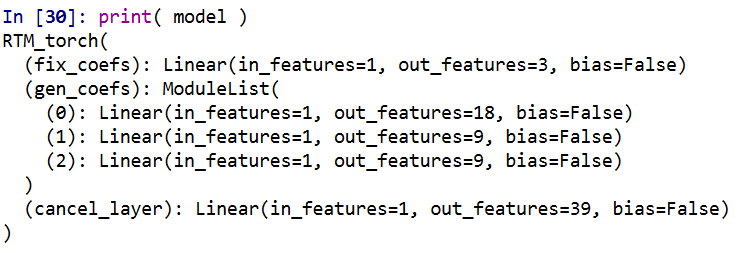The variable selection routine is very volatile – so if you have very correlated inputs, you can essentially swap one for the other and get near equivalent predictions. I often see folks who do RTM analyses say something along the lines of, “OK this RTM selected A, and this RTM selected B, so they are different effects in these two samples” (sometimes pre/post, other times comparing different areas, and other times different crime outcomes). I think this is probably wrong though to make that inference, as there is quite a bit of noise in the variable selection process (and the variable selection process itself precludes making inferences on the coefficients themselves). My deep learning example inherited the same problems. So if you change the initialized weights, it may end up selecting totally different inputs in the end. To get the variable selection routine to at least select the same crime generator variables in my tests, I do a burn in period in which I implement a random dropout scheme. So instead of the typical dropout, for every forward pass it does a random dropout to only select one variable randomly out of each crime generator set. After that converges, I then use a pruning layer to only pick the coefficient that has the largest effect, and again do a large set of iterations to make sure the results converged. So different means but same ends to the typical RTM steps 4 and 5 above. I also have like I said a ReLU transformation after each layer, so the crime generator variables are always positive, any negative effects will be pruned out. One thing that is nice about deep learning is that it can be quite fast. Here each of these 10,000 iteration sets take less than a minute on my desktop with a GPU. (I’ve been prototyping models with more parameters and more observations at work on my laptop with just a CPU that only take like 10 to 20 minutes). ###################################################### #Burn in part, random dropout for t in range(10000): #Forward pass y_pred = model(x=x_ten) #Loss loss_insample = criterion(y_pred, y_ten) optimizer.zero_grad() loss_insample.backward(retain_graph=True) optimizer.step() if t % 1000 == 0: print(f'loss: {loss_insample.item()}' ) #Switching to pruning all but the largest effects model.l1_prune() for t in range(10000): #Forward pass y_pred = model(x=x_ten, mask_type=None, cancel=False) #Loss loss_insample = criterion(y_pred, y_ten) optimizer.zero_grad() loss_insample.backward(retain_graph=True) optimizer.step() if t % 1000 == 0: print(f'loss: {loss_insample.item()}' ) print( model.coef_df(nm_li=full_set, cancel=False) ) ###################################################### And this prints out the results (as incident rate ratios), so you can see it selected 50 meters alcohol kernel density, 50 meters distance to the nearest metro station, and kernel density for 311 calls with an 800 meter bandwidth.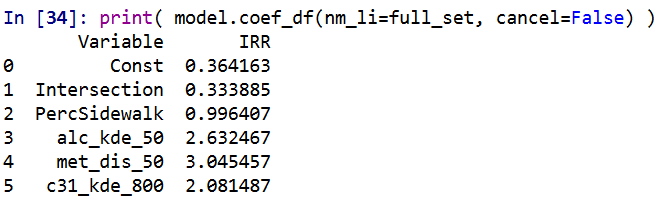I have in the code another example model when using a different seed. So testing out on around 5 different seeds it always selected these same distance/density variables, but the coefficients are slightly different each time. Here is an example from setting the seed to 12.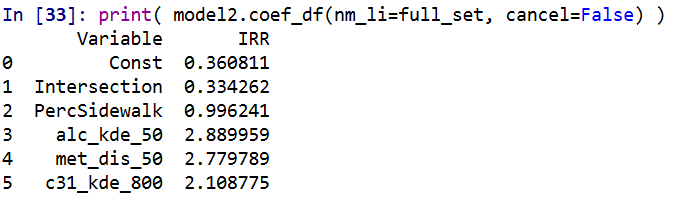These models are nothing to brag about, using the typical z-score the predictions and set the threshold to above 2, the PAI is only around 3 (both for in-sample 2011 and out of sample 2012 is slightly lower). It is a tough prediction task – the mean number of violent crimes per street unit per year is only 0.3. Violent crime is fortunately very rare! But with only three different risk variables, we can do a quick conjunctive analysis, and look at the areas of overlap. ###################################################### #Adding model 1 predictions back into the dataset pred_mod1 = pd.Series(model(x=x_ten, mask_type=None, cancel=False).exp().detach().numpy()) crime_data['Pred_M1'] = pred_mod1 #Check out the areas of overlapping risk mod1_coef = model.coef_df(nm_li=full_set, cancel=False) risk_vars = list(set(mod1_coef['Variable']) - set(const_li)) conj_set = crime_data.groupby(risk_vars, as_index=False)['Const','Pred_M1','Viol_2012'].sum() print(conj_set) ###################################################### In this table Const is the total number of street units selected, Pred_M1 is the expected number of crimes via Model 1, and then I show how well it conforms to the predictions out of sample 2012. So you can see in the aggregate the predictions are not too far off. There only ends up being one street unit that overlaps for all three risk factors in the study area.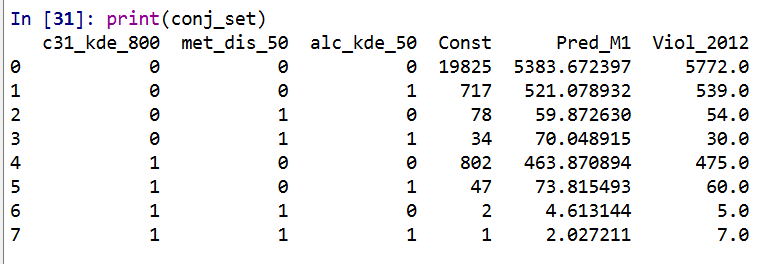I believe the predictions would be better if I included more crime generator variables. But ultimately the nature of how RTM works it trades off accuracy for simple models. Which is fair – it helps to ease the nature of how a police department (or some other entity) responds to the predictions. But this trade off results in predictions that don’t fare as well compared with more complicated models. For example I show (with Wouter Steenbeek) that random forests do much better than RTM. To make those models more interpretable we did local decompositions for hot spots, so say this hot spot is 30% alcohol outlets, 20% nearby apartments, etc. So there is no doubt more extensions for RTM you could do in a deep learning framework, but they will likely always result in more complicated and less interpretable models. Also here I don’t think this code will be better than the traditional RTM folks, the only major benefit of this code is it will run faster – minutes instead of overnight for most jobs. # Notes on making scatterplots in matplotlib and seaborn Many of my programming tips, like my notes for making Leaflet maps in R or margins plots in Stata, I’ve just accumulated doing projects over the years. My current workplace is a python shop though, so I am figuring it out all over for some of these things in python. I made some ugly scatterplots for a presentation the other day, and figured it would be time to spend alittle time making some notes on making them a bit nicer. For prior python graphing post examples, I have: For this post, I am going to use the same data I illustrated with SPSS previously, a set of crime rates in Appalachian counties. Here you can download the dataset and the python script to follow along. # Making scatterplots using matplotlib So first for the upfront junk, I load my libraries, change my directory, update my plot theme, and then load my data into a dataframe crime_dat. I technically do not use numpy in this script, but soon as I take it out I’m guaranteed to need to use np. for something! ################################################################ import pandas as pd import numpy as np import os import matplotlib import matplotlib.pyplot as plt import seaborn as sns my_dir = r'C:\Users\andre\OneDrive\Desktop\big_scatter' os.chdir(my_dir) andy_theme = {'axes.grid': True, 'grid.linestyle': '--', 'legend.framealpha': 1, 'legend.facecolor': 'white', 'legend.shadow': True, 'legend.fontsize': 14, 'legend.title_fontsize': 16, 'xtick.labelsize': 14, 'ytick.labelsize': 14, 'axes.labelsize': 16, 'axes.titlesize': 20, 'figure.dpi': 100} matplotlib.rcParams.update(andy_theme) crime_dat = pd.read_csv('Rural_appcrime_long.csv') ################################################################ First, lets start from the base scatterplot. After defining my figure and axis objects, I add on the ax.scatter by pointing the x and y’s to my pandas dataframe columns, here Burglary and Robbery rates per 100k. You could also instead of starting from the matplotlib objects start from the pandas dataframe methods (as I did in my prior histogram post). I don’t have a good reason for using one or the other. Then I set the axis grid lines to be below my points (is there a way to set this as a default?), and then I set my X and Y axis labels to be nicer than the default names. ################################################################ #Default scatterplot fig, ax = plt.subplots(figsize=(6,4)) ax.scatter(crime_dat['burg_rate'], crime_dat['rob_rate']) ax.set_axisbelow(True) ax.set_xlabel('Burglary Rate per 100,000') ax.set_ylabel('Robbery Rate per 100,000') plt.savefig('Scatter01.png', dpi=500, bbox_inches='tight') plt.show() ################################################################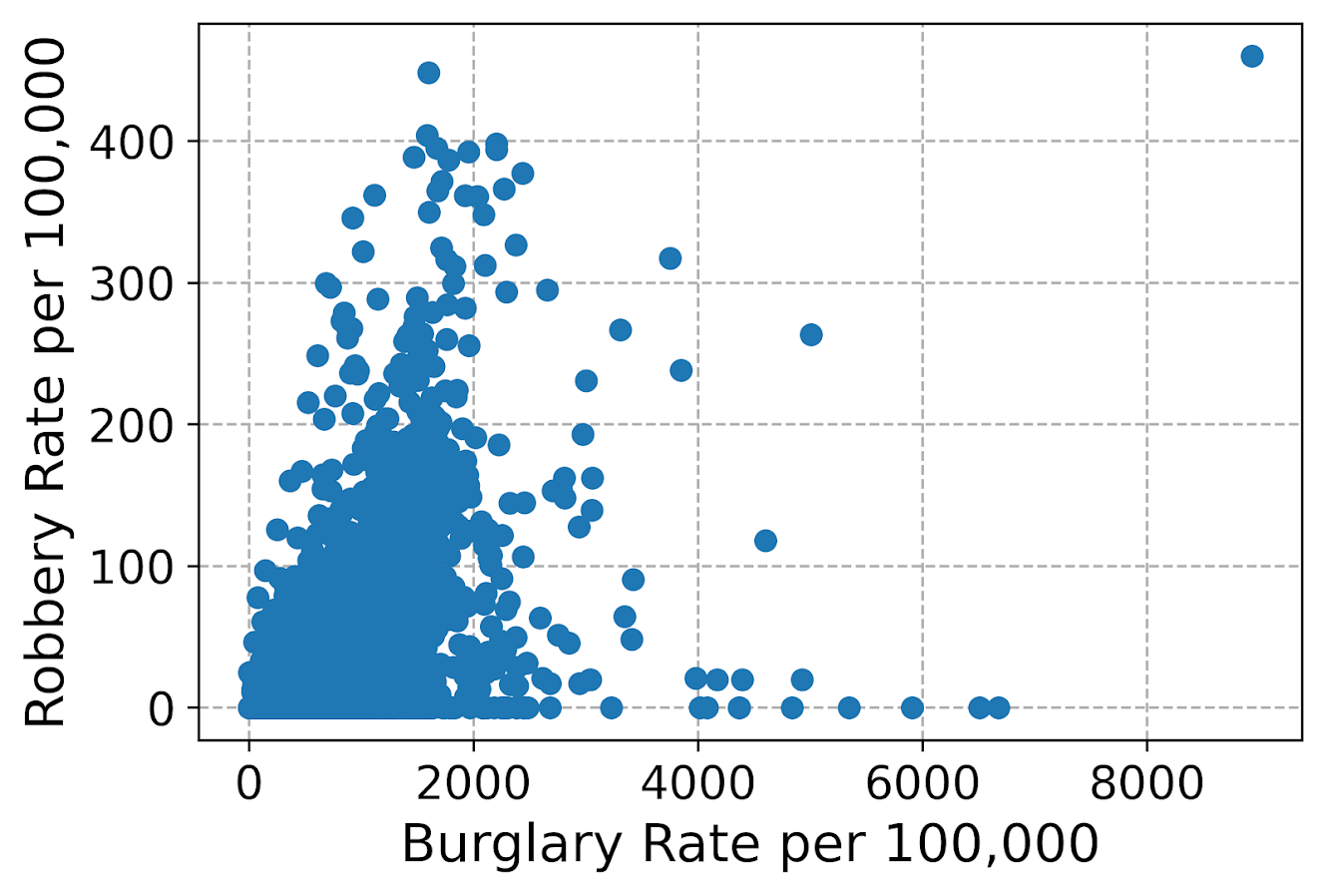You can see here the default point markers, just solid blue filled circles with no outline, when you get a very dense scatterplot just looks like a solid blob. I think a better default for scatterplots is to plot points with an outline. Here I also make the interior fill slightly transparent. All of this action is going on in the ax.scatter call, all of the other lines are the same. ################################################################ #Making points have an outline and interior fill fig, ax = plt.subplots(figsize=(6,4)) ax.scatter(crime_dat['burg_rate'], crime_dat['rob_rate'], c='grey', edgecolor='k', alpha=0.5) ax.set_axisbelow(True) ax.set_xlabel('Burglary Rate per 100,000') ax.set_ylabel('Robbery Rate per 100,000') plt.savefig('Scatter02.png', dpi=500, bbox_inches='tight') plt.show() ################################################################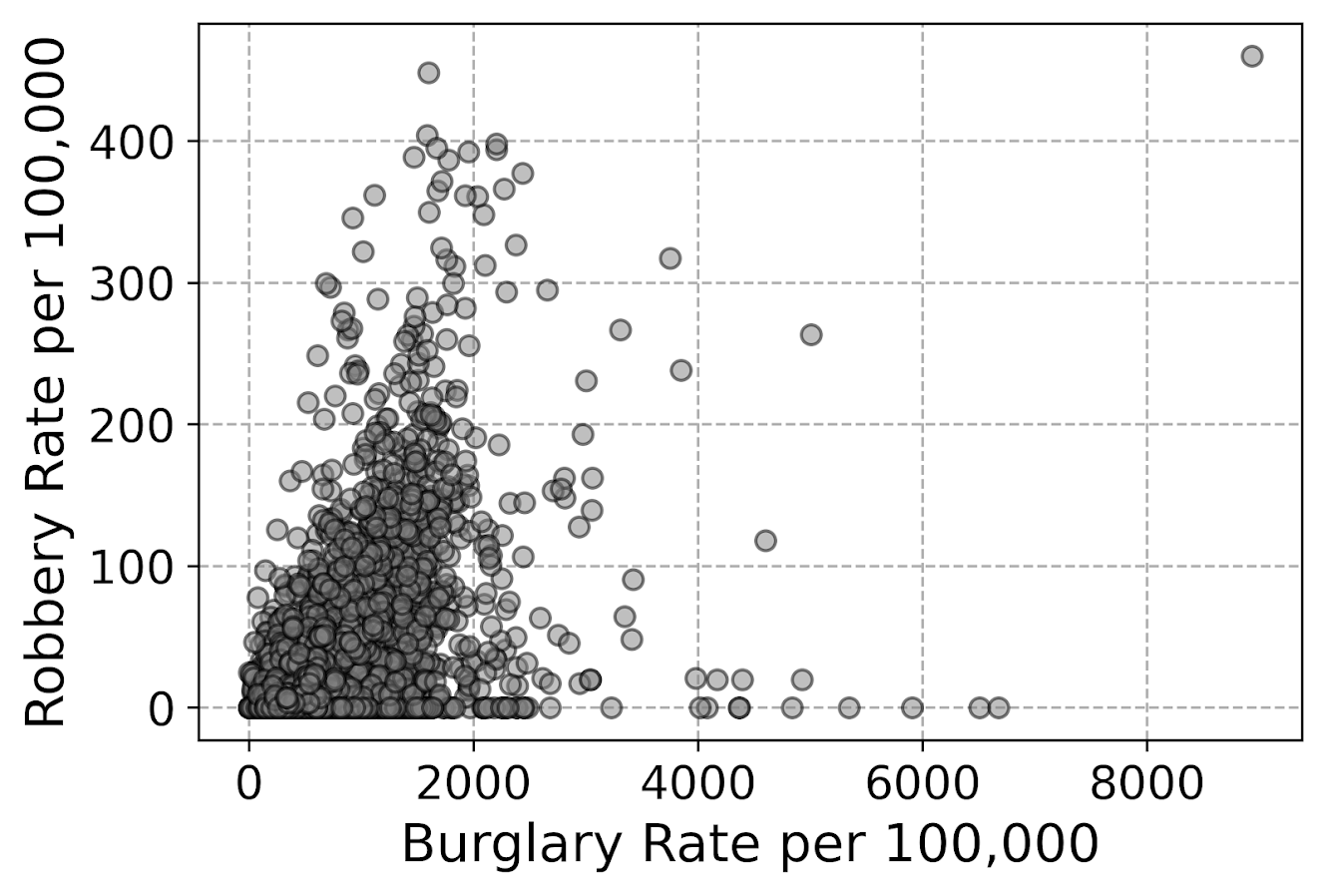So that is better, but we still have quite a bit of overplotting going on. Another quick trick is to make the points smaller and up the transparency by setting alpha to a lower value. This allows you to further visualize the density, but then makes it a bit harder to see individual points – if you started from here you might miss that outlier in the upper right. Note I don’t set the edgecolor here, but if you want to make the edges semitransparent as well you could do edgecolor=(0.0, 0.0, 0.0, 0.5), where the last number of is the alpha transparency tuner. ################################################################ #Making the points small and semi-transparent fig, ax = plt.subplots(figsize=(6,4)) ax.scatter(crime_dat['burg_rate'], crime_dat['rob_rate'], c='k', alpha=0.1, s=4) ax.set_axisbelow(True) ax.set_xlabel('Burglary Rate per 100,000') ax.set_ylabel('Robbery Rate per 100,000') plt.savefig('Scatter03.png', dpi=500, bbox_inches='tight') plt.show() ################################################################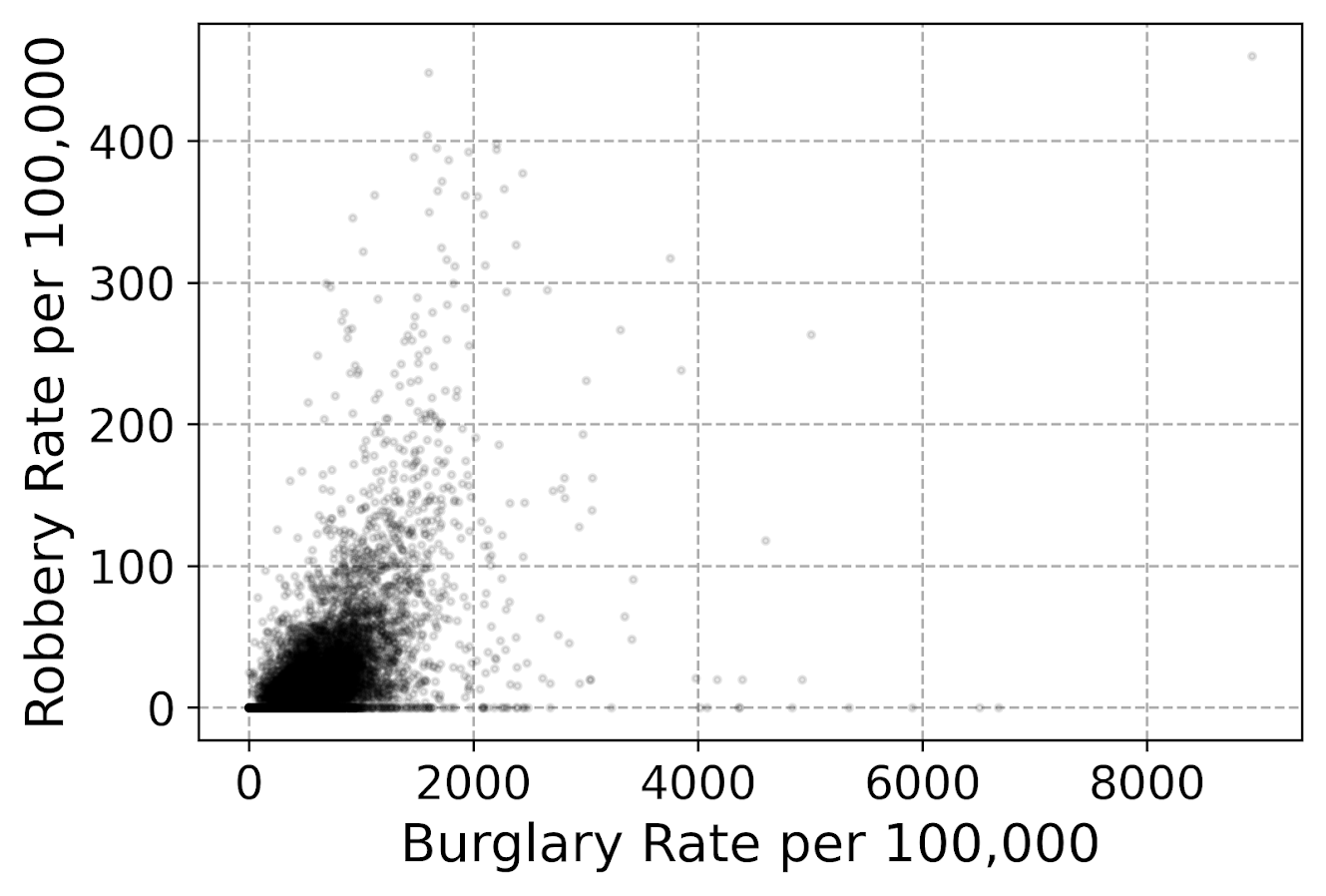This dataset has around 7.5k rows in it. For most datasets of anymore than a hundred points, you often have severe overplotting like you do here. One way to solve that problem is to bin observations, and then make a graph showing the counts within the bins. Matplotlib has a very nice hexbin method for doing this, which is easier to show than explain. ################################################################ #Making a hexbin plot fig, ax = plt.subplots(figsize=(6,4)) hb = ax.hexbin(crime_dat['burg_rate'], crime_dat['rob_rate'], gridsize=20, edgecolors='grey', cmap='inferno', mincnt=1) ax.set_axisbelow(True) ax.set_xlabel('Burglary Rate per 100,000') ax.set_ylabel('Robbery Rate per 100,000') cb = fig.colorbar(hb, ax=ax) plt.savefig('Scatter04.png', dpi=500, bbox_inches='tight') plt.show() ################################################################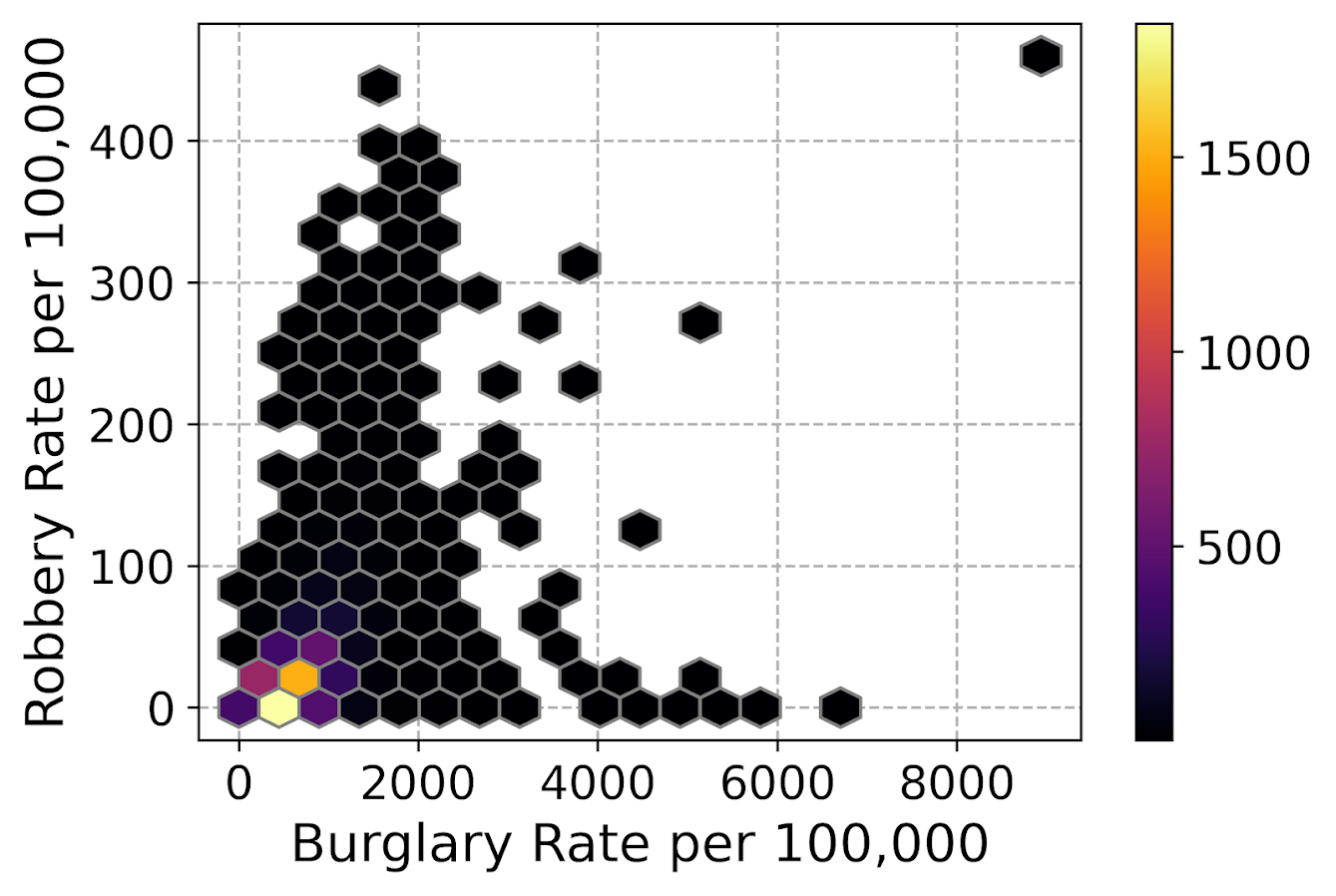So for the hexbins I like using the mincnt=1 option, as it clearly shows areas with no points, but then you can still spot the outliers fairly easy. (Using white for the edge colors looks nice as well.) You may be asking, what is up with that outlier in the top right? It ends up being Letcher county in Kentucky in 1983, which had a UCR population estimate of only 1522, but had a total of 136 burglaries and 7 robberies. This could technically be correct (only some local one cop town reported, and that town does not cover the whole county), but I’m wondering if this is a UCR reporting snafu. It is also a good use case for funnel charts. I debated on making some notes here about putting in text labels, but will hold off for now. You can add in text by using ax.annotate fairly easy by hand, but it is hard to automate text label positions. It is maybe easier to make interactive graphs and have a tooltip, but that will need to be another blog post as well. # Making scatterplots using seaborn The further examples I show are using the seaborn library, imported earlier as sns. I like using seaborn to make small multiple plots, but it also has a very nice 2d kernel density contour plot method I am showing off. Note this does something fundamentally different than the prior hexbin chart, it creates a density estimate. Here it looks pretty but creates a density estimate in areas that are not possible, negative crime rates. (There are ways to prevent this, such as estimating the KDE on a transformed scale and retransforming back, or reflecting the density back inside the plot would probably make more sense here, ala edge weighting in spatial statistics.) Here the only other things to note are used filled contours instead of just the lines, I also drop the lowest shaded area (I wish I could just drop areas of zero density, note dropping the lowest area drops my outlier in the top right). Also I have a tough go of using the default bandwidth estimators, so I input my own. ################################################################ #Making a contour plot using seaborn g = sns.kdeplot(crime_dat['burg_rate'], crime_dat['rob_rate'], shade=True, cbar=True, gridsize=100, bw=(500,50), cmap='plasma', shade_lowest=False, alpha=0.8) g.set_axisbelow(True) g.set_xlabel('Burglary Rate per 100,000') g.set_ylabel('Robbery Rate per 100,000') plt.savefig('Scatter05.png', dpi=500, bbox_inches='tight') plt.show() ################################################################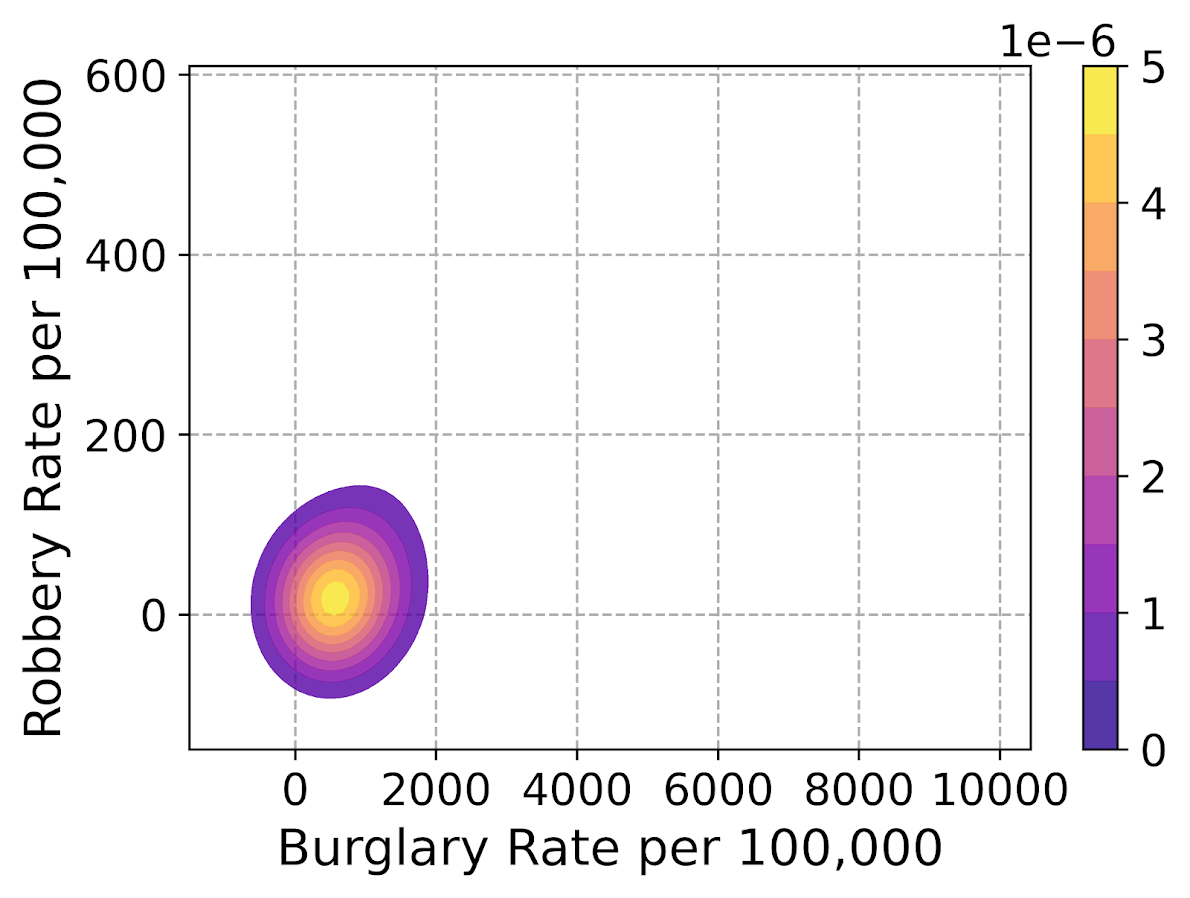So far I have not talked about the actual marker types. It is very difficult to visualize different markers in a scatterplot unless they are clearly separated. So although it works out OK for the Iris dataset because it is small N and the species are clearly separated, in real life datasets it tends to be much messier. So I very rarely use multiple point types to symbolize different groups in a scatterplot, but prefer to use small multiple graphs. Here is an example of turning my original scatterplot, but differentiating between different county areas in the dataset. It is a pretty straightforward update using sns.FacetGrid to define the group, and then using g.map. (There is probably a smarter way to set the grid lines below the points for each subplot than the loop.) ################################################################ #Making a small multiple scatterplot using seaborn g = sns.FacetGrid(data=crime_dat, col='subrgn', col_wrap=2, despine=False, height=4) g.map(plt.scatter, 'burg_rate', 'rob_rate', color='grey', s=12, edgecolor='k', alpha=0.5) g.set_titles("{col_name}") for a in g.axes: a.set_axisbelow(True) g.set_xlabels('Burglary Rate per 100,000') g.set_ylabels('Robbery Rate per 100,000') plt.savefig('Scatter06.png', dpi=500, bbox_inches='tight') plt.show() ################################################################And then finally I show an example of making a small multiple hexbin plot. It is alittle tricky, but this is an example in the seaborn docs of writing your own sub-plot function and passing that. To make this work, you need to pass an extent for each subplot (so the hexagons are not expanded/shrunk in any particular subplot). You also need to pass a vmin/vmax argument, so the color scales are consistent for each subplot. Then finally to add in the color bar I just fiddled with adding an axes. (Again there is probably a smarter way to scoop up the plot coordinates for the last plot, but here I just experimented till it looked about right.) ################################################################ #Making a small multiple hexbin plot using seaborn #https://github.com/mwaskom/seaborn/issues/1860 #https://stackoverflow.com/a/31385996/604456 def loc_hexbin(x, y, **kwargs): kwargs.pop("color", None) plt.hexbin(x, y, gridsize=20, edgecolor='grey', cmap='inferno', mincnt=1, vmin=1, vmax=700, **kwargs) g = sns.FacetGrid(data=crime_dat, col='subrgn', col_wrap=2, despine=False, height=4) g.map(loc_hexbin, 'burg_rate', 'rob_rate', edgecolors='grey', extent=[0, 9000, 0, 500]) g.set_titles("{col_name}") for a in g.axes: a.set_axisbelow(True) #This goes x,y,width,height cax = g.fig.add_axes([0.55, 0.09, 0.03, .384]) plt.colorbar(cax=cax, ax=g.axes) g.set_xlabels('Burglary Rate per 100,000') g.set_ylabels('Robbery Rate per 100,000') plt.savefig('Scatter07.png', dpi=500, bbox_inches='tight') plt.show() ################################################################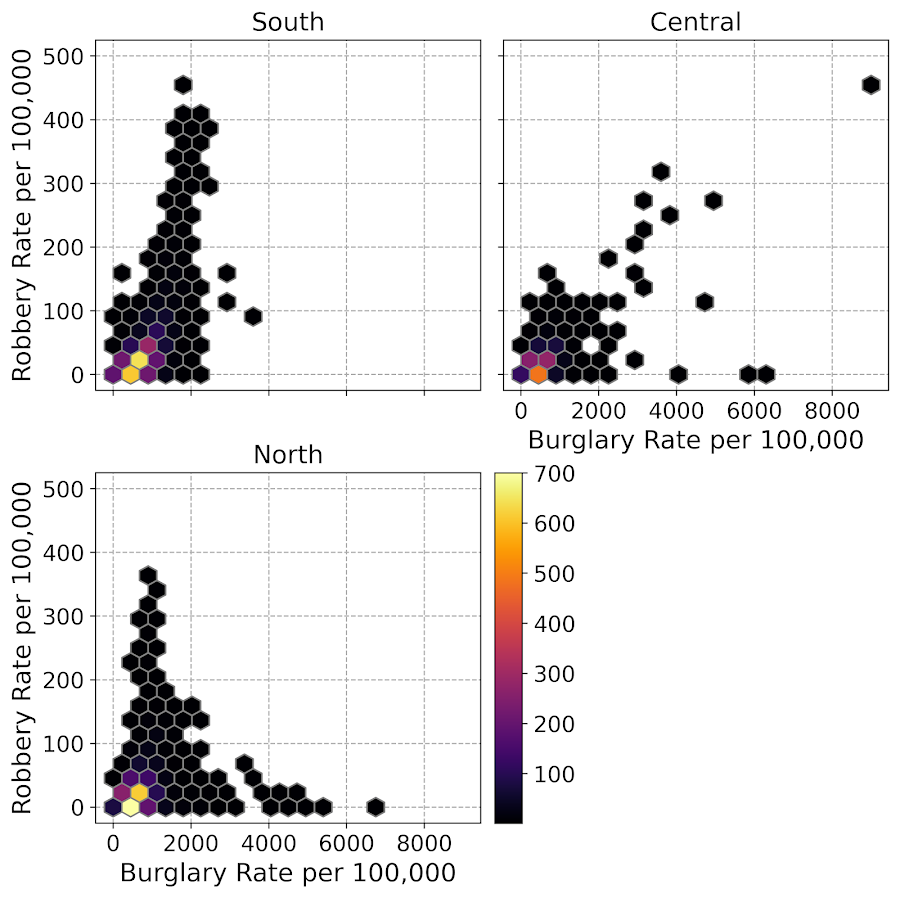Another common task with scatterplots is to visualize a smoother, e.g. E[Y|X] the expected mean of Y conditional on X, or you could do any other quantile, etc. That will have to be another post though, but for examples I have written about previously I have jittering 0/1 data, and visually weighted regression. # Notes on making Leaflet maps in R The other day I wrote a blog post for crimrxiv about posting interactive graphics on their pre-print sharing service. I figured it would be good to share my notes on making interactive maps, and to date I’ve mostly created these using the R leaflet library. The reason I like these interactive maps is they allow you to zoom in and look at hot spots of crime. With the slippy base maps you can then see, oh OK this hot spot is by a train station, or an apartment complex, etc. It also allows you to check out specific data labels via pop-ups as I will show. I’m using data from my paper on creating cost of crime weighted hot spots in Dallas (that will be forthcoming in Police Quarterly soonish). But I have posted a more direct set of replicating code for the blog post here. # R Code So first for the R libraries I am using, I also change the working directory to where I have my data located on my Windows machine. ########################################################## #This code creates a nice leaflet map of my DBSCAN areas library(rgdal) #read in shapefiles library(sp) #spatial objects library(leaflet) #for creating interactive maps library(htmlwidgets) #for exporting interactive maps #will need to change baseLoc if replicating on your machine baseLoc <- "D:\\Dropbox\\Dropbox\\Documents\\BLOG\\leaflet_R_examples\\Analysis" setwd(baseLoc) ########################################################## Second, I read in my shapefiles using the rgdal library. This is important, as it includes the projection information. To plot the spatial objects on a slippy map they need to be in the Web Mercator projection (or technically no projection, just a coordinate reference system for the globe). As another trick I like with these basemaps, for the outlined area (the Dallas boundary here), it is easier to plot as a line spatial object, as opposed to an empty filled polygon. You don’t need to worry about the order of the layers as much that way. ########################################################## #Get the boundary data and DBSCAN data boundary <- readOGR(dsn="Dallas_MainArea_Proj.shp",layer="Dallas_MainArea_Proj") dbscan_areas <- readOGR(dsn="db_scan.shp",layer="db_scan") #Now convert to WGS DalLatLon <- spTransform(boundary,CRS("+init=epsg:4326")) DallLine <- as(DalLatLon, 'SpatialLines') #Leaflet useful for boundaries to be lines instead of areas dbscan_LatLon <- spTransform(dbscan_areas,CRS("+init=epsg:4326") ) #Quick and Dirty plot to check projections are OK plot(DallLine) plot(dbscan_LatLon,add=TRUE,col='blue') ########################################################## Next part, I have a custom function I have made to make pop-up labels for these leaflet maps. First I need to read in a table with the data info for the hot spot areas and merge that into the spatial object. Then the way my custom function works is I pass it the dataset, then I have arguments for the variables I want, and the way I want them labeled. The function does the work of making the labels bolded and putting in line breaks into the HTML. (No doubt others have created nice libraries to do HTML tables/graphs inside the pop-ups that I am unaware of.) If you check out the final print statement, it shows the HTML it built for one of the labels, <strong>ID: </strong>1<br><strong>$ (Thousands): </strong>116.9<br><strong>PAI: </strong>10.3<br><strong>Street Length (Miles): </strong>0.4

##########################################################
#Function for labels

dbscan_stats <- crime_stats[crime_stats$type == 'DBSCAN',] dbscan_stats$clus_id <- as.numeric(dbscan_stats$AreaStr) #because factors=False! #merge into the dbscan areas dbscan_LL <- merge(dbscan_LatLon,dbscan_stats) LabFunct <- function(data,vars,labs){ n <- length(labs) add_lab <- paste0("<strong>",labs,"</strong>",data[,vars]) for (i in 2:n){ add_lab <- paste0(add_lab,"<br><strong>",labs[i],"</strong>",data[,vars[i]]) } return(add_lab) } #create labels vs <- c('AreaStr', 'val_th', 'PAI_valth_len', 'LenMile') #Lazy, so just going to round these values for (v in vs[-1]){ dbscan_LL@data[,v] <- round(dbscan_LL@data[,v],1) } lb <- c('ID: ','$ (Thousands): ','PAI: ','Street Length (Miles): ')
diss_lab <- LabFunct(dbscan_LL@data, vs, lb)

print(diss_lab) #showing off just one
##########################################################

Now finally onto the hotspot map. This is a bit to chew over, so I will go through bit-by-bit.

##########################################################
HotSpotMap <- leaflet() %>%
addProviderTiles(providers$OpenStreetMap, group = "Open Street Map") %>% addProviderTiles(providers$CartoDB.Positron, group = "CartoDB Lite") %>%
addPolylines(data=DallLine, color='black', weight=4, group="Dallas Boundary") %>%
addPolygons(data=dbscan_LL,color = "blue", weight = 2, opacity = 1.0,
fillOpacity = 0.5, group="DBSCAN Areas",popup=diss_lab,
highlight = highlightOptions(weight = 5,bringToFront = TRUE)) %>%
addLayersControl(baseGroups = c("Open Street Map","CartoDB Lite"),
overlayGroups = c("Dallas Boundary","DBSCAN Areas"),
options = layersControlOptions(collapsed = FALSE))  %>%
addScaleBar(position = "bottomleft", options = scaleBarOptions(maxWidth = 100,
imperial = TRUE, updateWhenIdle = TRUE))

HotSpotMap #this lets you view interactively

#or save to a HTML file to embed in webpage
saveWidget(HotSpotMap,"HotSpotMap.html", selfcontained = TRUE)
##########################################################

First I create the empty leaflet() object. Because I am superimposing multiple spatial layers, I don’t worry about setting the default spatial layer. Second, I add in two basemap providers, OpenStreetMap and the grey scale CartoDB positron. Positron is better IMO for visualizing global data patterns, but the open street map is better for when you zoom in and want to see exactly what is around a hot spot area. Note when adding in a layer, I give it a group name. This allows you to later toggle which provider you want via a basegroup in the layers control.

Next I add in the two spatial layers, the Dallas Boundary lines and then the hot spots. For the DBSCAN hot spots, I include a pop-up diss_lab for the dbscan hot spot layer. This allows you to click on the polygon, and you get the info I stuffed into that label vector earlier. The HTML is to make it print nicely.

Finally then I add in a layers control, so you can toggle layers on/off. Basegroups mean that only one of the options can be selected, it doesn’t make sense to have multiple basemaps selected. Overlay you can toggle on/off as needed. Here the overlay doesn’t matter much due to the nature of the map, but if you have many layers (e.g. a hot spot map and a choropleth map of demographics) being able to toggle the layers on/off helps a bit more.

Then as a final touch I add in a scale bar (that automatically updates depending on the zoom level). These aren’t my favorite with slippy maps, as I’m not even 100% sure what location the scale bar refers to offhand (the center of the map? Or literally where the scale bar is located?) But when zoomed into smaller areas like a city I guess it is not misleading.

Here is a screenshot of this created map zoomed out to the whole city using the Positron grey scale base map. So it is tough to visualize the distribution of hot spots from this. If I wanted to do that in a static map I would likely just plot the hot spot centroids, and then make the circles bigger for areas that capture more crime.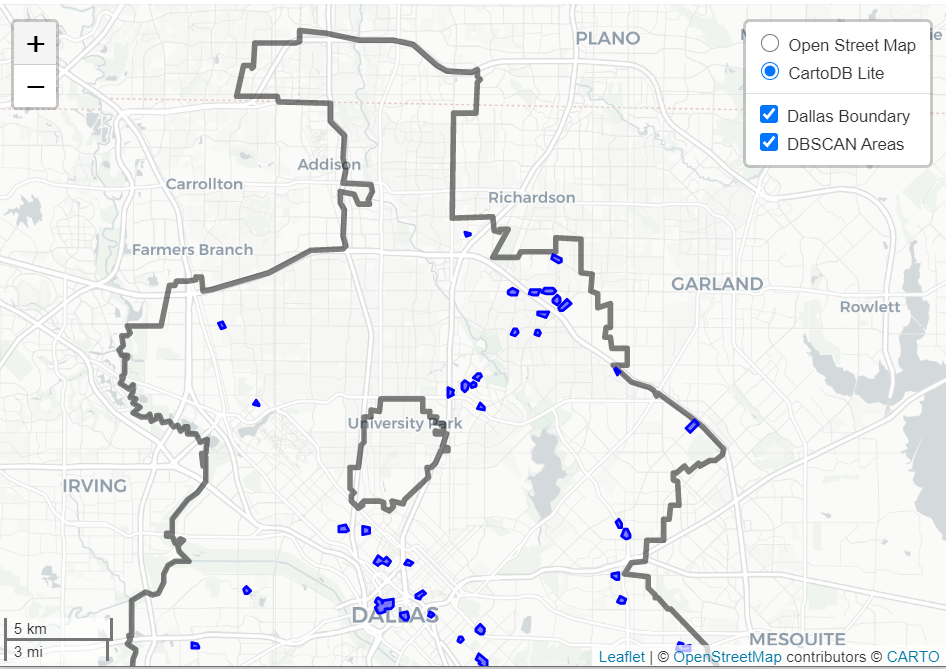But since we can zoom in, here is another screenshot zoomed in using the OpenStreetMap basemap, and also illustrating what my pop-up labels look like.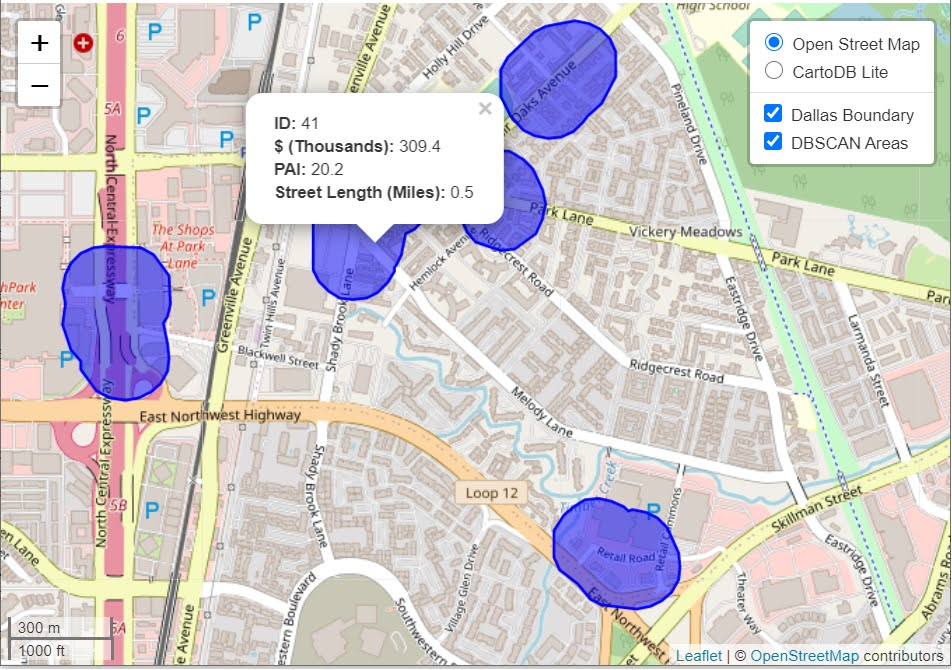I’m too lazy to post this exact map, but it is very similar to one I posted for my actual hot spots paper if you want to check it out directly. I host it on GitHub for free.

Here I did not show how to make a choropleth map, but Jacob Kaplan in his R book has a nice example of that. And in the future I will have to update this to show how to do the same thing in python using the Folium library. I used Folium in this blog post if you want to dig into an example though for now.

# Some more examples

For some other examples of what is possible in Leaflet maps in R, here are some examples I made for my undergrad Communities and Crime class. I had students submit prediction assignments (e.g. predict the neighborhood with the most crime in Dallas, predict the street segment in Oak Cliff with the most violent crime, predict the bar with the most crimes nearby, etc.) I would then show the class the results, as well as where other students predicted. So here are some screen shots of those maps.

Choropleth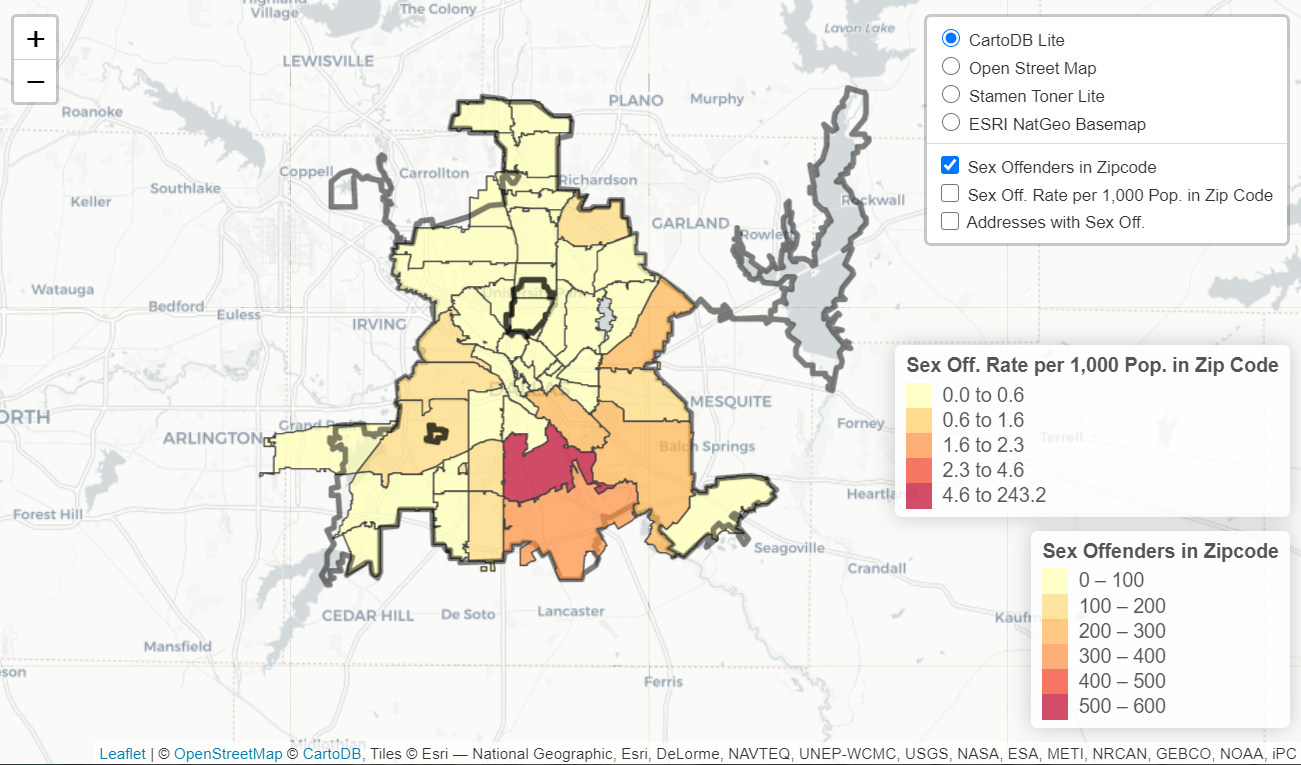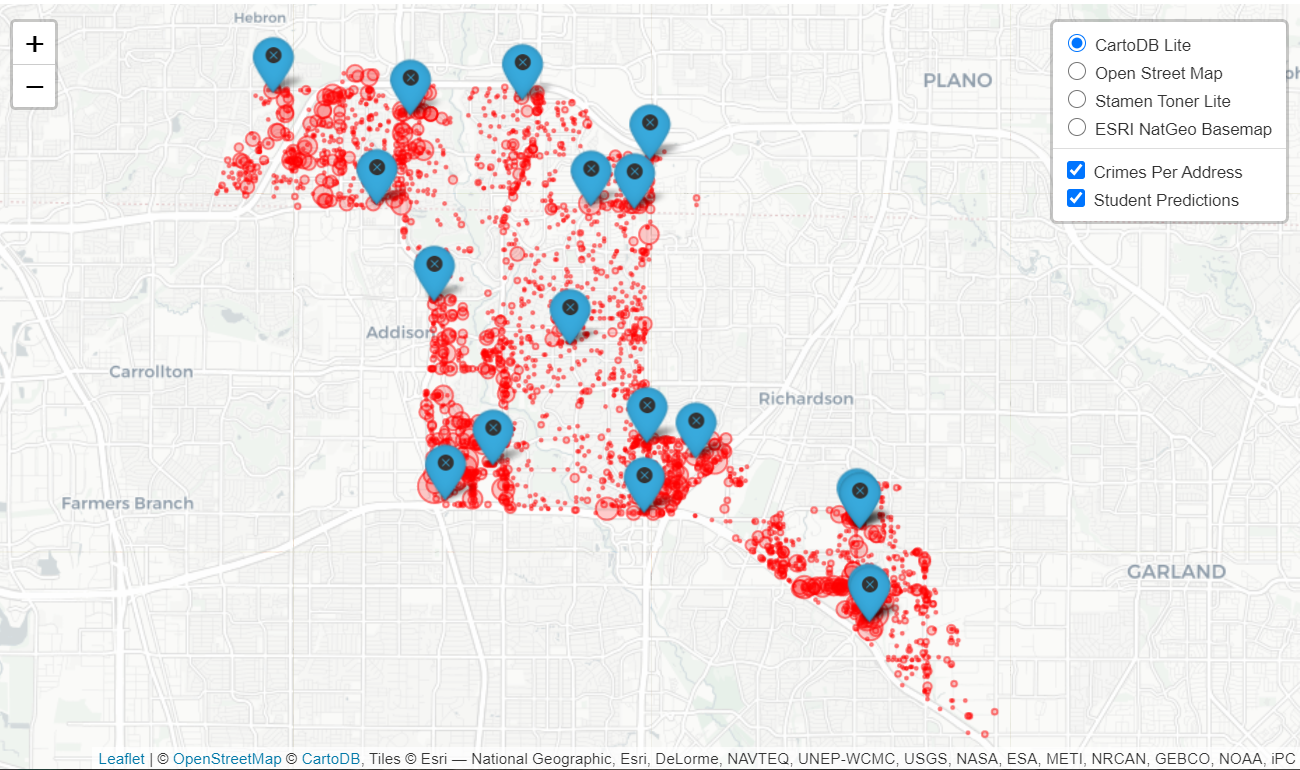Street Segment Viz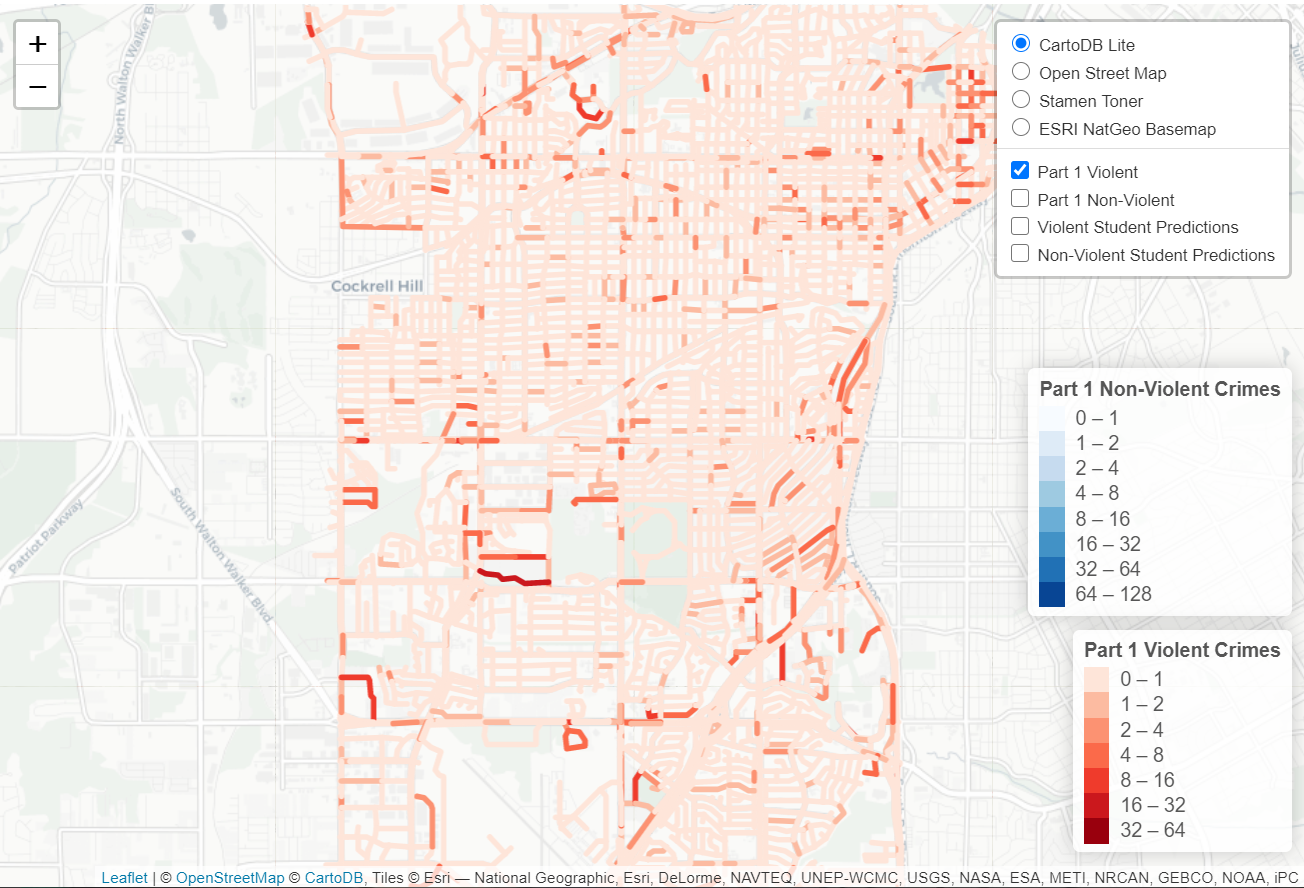# Creating high crime sub-tours

I was nerdsniped a bit by this paper, Targeting Knife-Enabled Homicides For Preventive Policing: A Stratified Resource Allocation Model by Vincent Hariman and Larry Sherman (HS from here on).

It in, HS attempt to define a touring schedule based on knife crime risk at the lower super output area in London. So here are the identified high risk areas: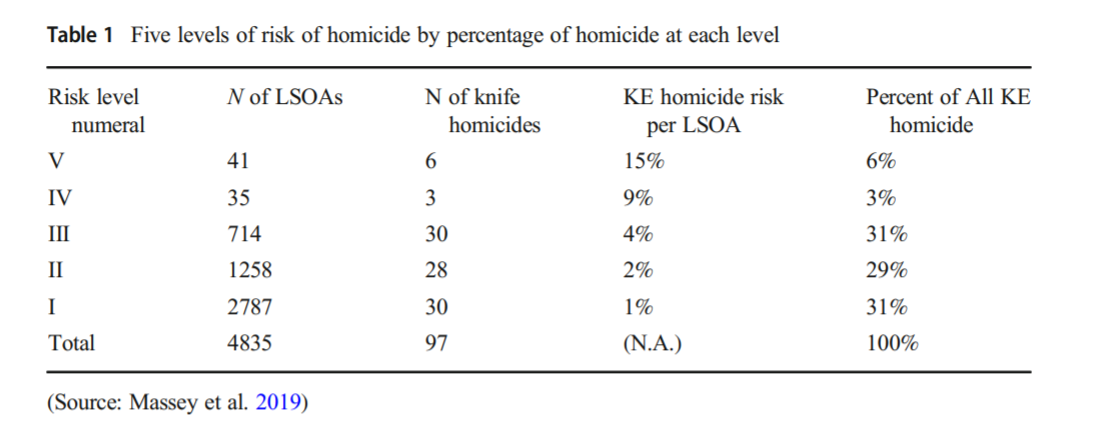And here are HS’s suggested hot spot tours schedule.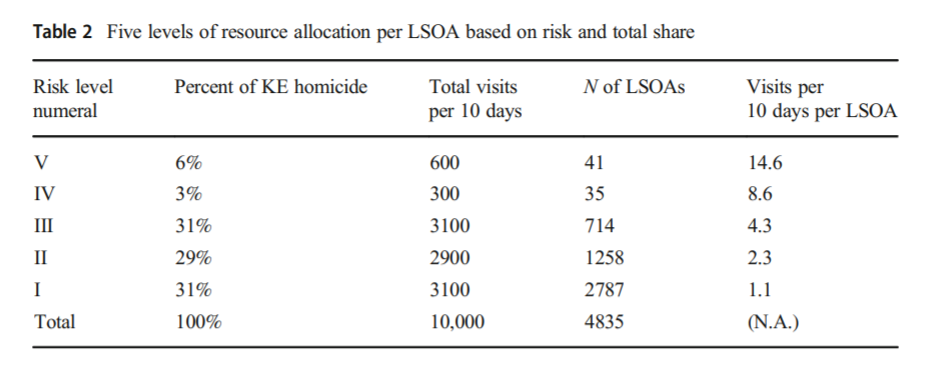This is ad-hoc, but an admirable attempt to figure out a reasonable schedule. As you can see in their tables, the ‘high’ knife crime risk areas still only have a handful of homicides, so if reducing homicides is the objective, this program is a bit dead in the water (I’ve written about the lack of predictive ability of the model here).

I don’t think defining tours to visit everywhere makes sense, but I do think a somewhat smaller in scope question, how to figure out geographically informed tours for hot spot areas does. So instead of the single grid cell target ala PredPol, pick out multiple areas to visit for hot spots. (I don’t imagine the 41 LSOA areas are geographically contiguous either, e.g. it would make more sense to pick a tour for areas connected than for areas very far apart.)

Officers don’t tend to like single tiny areas either really, and I think it makes more sense to widen the scope a bit. So here is my attempt to figure those reasonable tours out.

# Defining the Problem

The way I think about that problem is like this, look at the hypothetical diagram below. We have two choices for the hot spot location we are targeting, where the crime counts for locations are noted in the text label.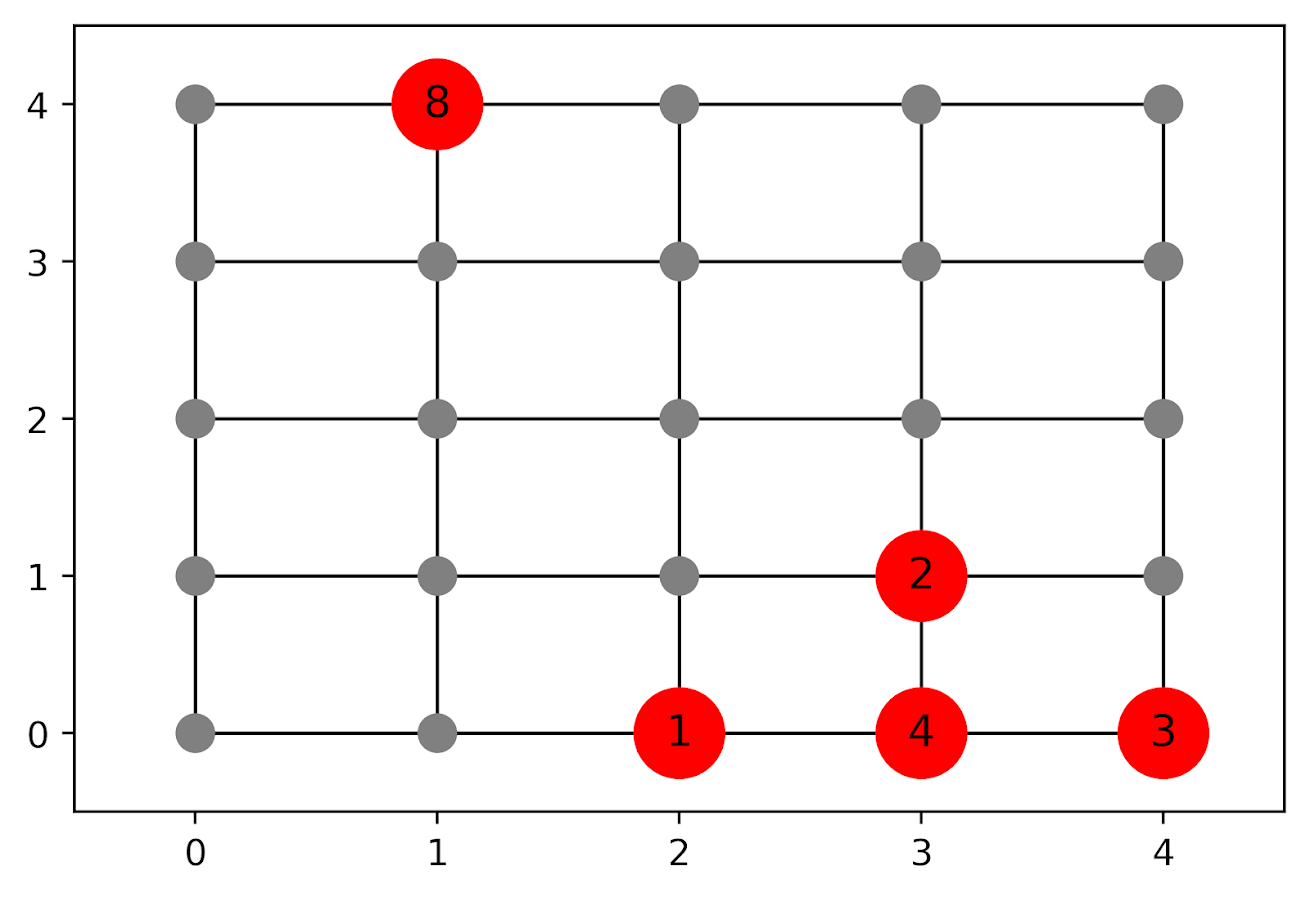In the select the top hot spot (e.g. PredPol) approach, you would select the singlet grid cell in the top left, it is the highest intensity. We have another choice though, the more spread out hot spot in the lower right. Even though it is a lower density, it ends up capturing more crime overall.

I subsequently formulated an integer linear program to try to tackle the problem of finding good sub-tours through the graph that cumulatively capture more crime. So with the above graph, if I select two subtours, I get the results as (where nodes are identified by their (x,y) position):

• ['Begin', (1, 4), 'End']
• ['Begin', (4, 0), (4, 1), (3, 1), (3, 0), (2, 0), 'End']

So it can select singlet areas if they are islands (the (1,4) area in the top left), but will grow to wind through areas. Also note that the way I have programmed this network, it doesn’t skip the zero area (4,1) (it needs to go through at least one in the bottom right unless it doubles back on itself).

I will explain the meaning of the begin and end nodes below in my description of the linear program. It ends up being sort of a mash-up of traveling salesman type vehicle routing and min cost max flow type problems.

# The Linear Program

The way I think about this problem formulation is like this: we have a directed graph, in which you can say, OK I start from location A, then can go to B, than go to C. In my set of decision variables, I have choices that look like this, where the first subscript denotes the from node, and the second subscript denotes the to node.

D_ab := node a -> node b
D_bc := node b -> node c

etc. In our subsequent linear program, the destination node is the node that we calculate our cumulative crime density statistics. So if node B had 10 crimes and 0.1 square kilometers, we would have a density of 100 crimes per square kilometer.

Now to make this formulation work, we need to add in a set of special nodes into our usual location network. These nodes I call ‘Begin’ and ‘End’ nodes (you may also call them source/sink nodes though). The begin nodes all look like this:

D_{begin},a
D_{begin},b
D_{begin},c

So you do that for every node in your network. Then you have End nodes as well, e.g.

D_a,{end}
D_b,{end}
D_c,{end}

In this formulation, since we are only concerned about the crime stats for the to node, not the from node, the Begin nodes just inherit the crime density stats from the original node data. For the end nodes though, you just set their objective value stats to zero (they are only relevant to define the constraints).

Now here is my linear program formulation:

Maximize
Sum [ D_ij ( CrimeDensity_j - DensityPenalty_j ) ]

Subject To:

1. Sum( D_in for each neighbor of n ) <= 1,
for each original node n
2. Sum( D_in for each neighbor of n ) =  Sum( D_ni for each neighbor of n ),
for each original node n
3. Sum( D_bi for each begin node ) = k routes
4. Sum( D_ie for each end node ) = k routes
5. Sum( D_ij + D_ji ) <= 1, for each unique i,j pair
6. D_ij is an element of {0,1}

Constraint 1 is a flow constraint. If a node has an incoming edge set to one, it cannot have any other incoming edge set to one (so a location can only be chosen once).

Constraint 2 is a constraint that says if an incoming node is selected, one of the outgoing edges needs to be selected.

Constraints 3 & 4 determine the number of k tours/routes to choose in the end. Since the begin/end nodes are special we have k routes going out of the begin nodes, and k routes going into the end nodes.

With just these constraints, you can still get micro-cycles I found. So something like, X -> Z -> X. Constraint 5 (for only the undirected edges) prevents this from happening.

Constraint 6 is just setting the decision variables to binary 0/1. So it is a mixed integer linear program.

The final thing to note is the objective function, I have CrimeDensity_j - DensityPenalty_j, so what exactly is DensityPenalty? This is a value that penalizes visiting areas that are below this threshold. Basically the way that this works is that, the density penalty sets an approximate threshold for the minimum density a tour should contain.

I suggest a default of a predictive accuracy index of 10. Where do I get 10 you ask? Weisburd’s law of crime concentration suggests 5% of the areas should contain 50% of the crime, which is a PAI of 0.5/0.05 = 10. In my example with DC data then I just calculate the actual density of crime per unit area that corresponds to a PAI of 10.

You can adjust this though, if you prefer smaller tours of higher crime density you would up the value. If you prefer longer tours decrease it.

This is the best way I could figure out how to trade off the idea of spreading out the targeted hot spot vs selecting the best areas. If you spread out you will ultimately have a lower density. This turns it into a soft objective penalty to try to keep the selected tours at a particular density threshold (and will scoop up better tours if they are available). For awhile I tried to figure out if I could maximize the PAI metric, but it is the case with larger areas the PAI will always go down, so you need to define the objective some other way.

This formulation I only consider linked nodes (unlike the usual traveling salesman in which it is a completely linked distance graph). That makes it much more manageable. In this formulation, if you have N as the number of nodes/areas, and E is the number of directed edges between those areas, we will then have:

• 2*N + E decision variables
• 2 + 2*N + E/2 constraints

Generally if you are doing directly connected areas in geographic networks (i.e. contiguity connections), you will have less than 8 (typically more like an average of 6) neighbors per each area. So in the case of the 4k London lower super output areas, if I chose tours I would guess it would end up being fewer than 2*4,000 + 8*4,000 = 40,000 decision variables, and then fewer than that constraints.

Since that is puny (and I would suggest doing this at a smaller geographic resolution) I tested it out on a harder network. I used the data from my dissertation, a network of 21,506 street units (both street segments and intersections) in Washington, D.C. The contiguity I use for these micro units is based on the Voronoi tessellation, so tends to have more neighbors than you would with a strictly road based network connectivity. Still in the end it ends up being a shade fewer than 200k decision variables and 110k constraints. So is a better test for in the wild whether the problem can be feasibly solved I think.

# Example with DC Data

Here I have posted the python code and data used for this analysis, I end up having a nice function that you just submit your network with the appropriate attributes and out pops the different tours.

So I end up doing examples of 4 and 8 subtours based on 2011 violent UCR crime data (agg assaults, robberies, and homicides, no rapes in the public data). I use for the penalty that PAI = 10 threshold, so it should limit tours to approximately that value. It only ends up taking 2 minutes for the model to converge for the 4 tours and less than 2.5 minutes for the 8 tours on my desktop. So it should be not a big problem to up the decision variables to more sub-areas and still be solvable in real life applications.

The area estimates are in square meters, hence the high numbers. But on the right you can see that each sub-tour has a PAI above 10.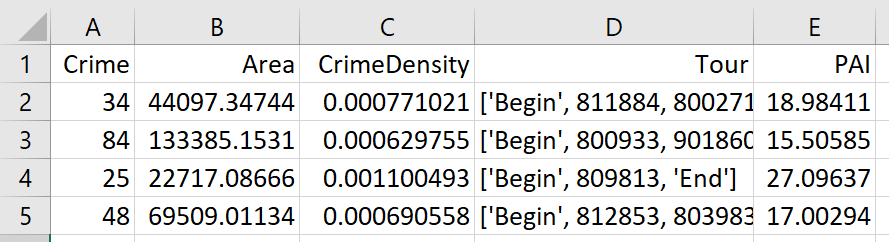Here is an interactive map for you to zoom into each 4 subtour example. Below is a screenshot of one of the subtours. You can see that since I have defined my connected areas in terms of Voronoi tessalations, they don’t exactly follow the street network.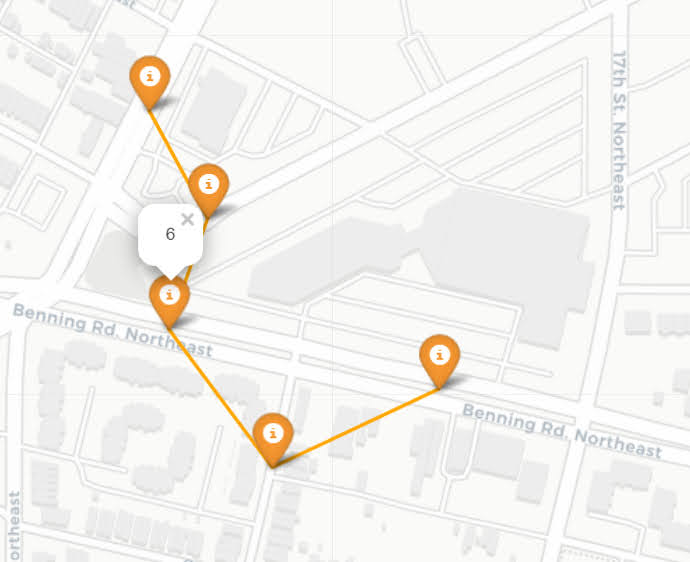For the 8 tour example, it ends up returning several zero tours, so it is not possible in this data to generate 8 sub-tours that meet that PAI >= 10 threshold.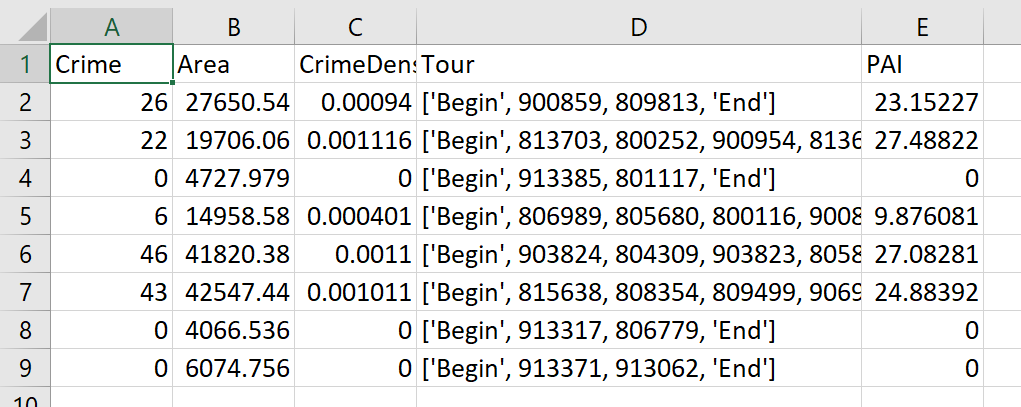You can see that it ends up being the tours have higher PAI values, but lower overall crime counts.

You may think, why does it not pick at least singlet areas with at least one crime? It ends up being that I weight areas here by their area (this formulation would be better with grid cells of equal area, so my objective function is technically Sum [ D_ij * w_j *( CrimeDensity_j - DensityPenalty_j ) ], where w_j is the percent of the total area (so the denominator in the PAI calculation). So it ends up picking areas that are the tiniest areas, as they result in the smallest penalty to the objective function (w_j is tiny). I think this is OK though in the end – I rather know that some of the tours are worthless.

You can also see I get one subtour that is just under the PAI 10 threshold. Again possible here, but should be only slightly below in the worst case scenario. The way the objective function works is that it is pretty tricky to pick out subtours below that PAI value but still have a positive contribution to the overall objective function.

# Future Directions

The main thing I wish I could do with the current algorithm (but can’t the way the linear program is set up), is to have minimum and maximum tour area/length constraints. I think I can maybe do this by adapting this code (I’m not sure how to do the penalties/objectives though). So if others have ideas let me know!

I admit that this may be overkill, and maybe just doing more typical crime clustering algorithms may be sufficient. E.g. doing DBSCAN hot spots like I did here.

But this is my best attempt shake at the problem for now!

# Using Steiner trees to select a subgraph of interest

This is just a quick blog post. A crime analyst friend the other day posed a network problem to me. They had a social network in which they had particular individuals of interest, and wanted to show just a subset of that graph that connected those key individuals. The motivation was for plotting – if you show the entire hairball it can become really difficult to uncover any relationships.

Here is an example gang network from this paper. I randomly chose 10 nodes to highlight (larger red circles), and you can see it is quite hairy. You often want to label the nodes for these types of graphs, but that becomes impossible with so many intertwined nodes.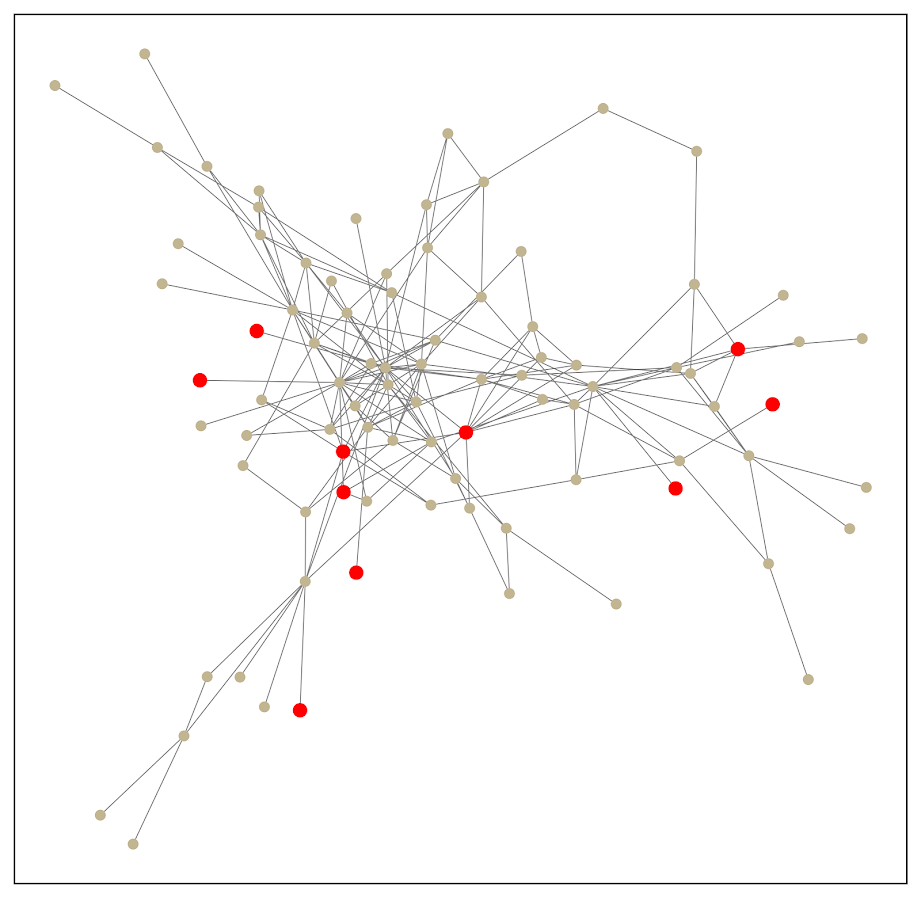One solution to select out a subgraph of the connected bits is to use a Steiner tree. Here is that graph after running the approximate Steiner tree algorithm in networkx (in python).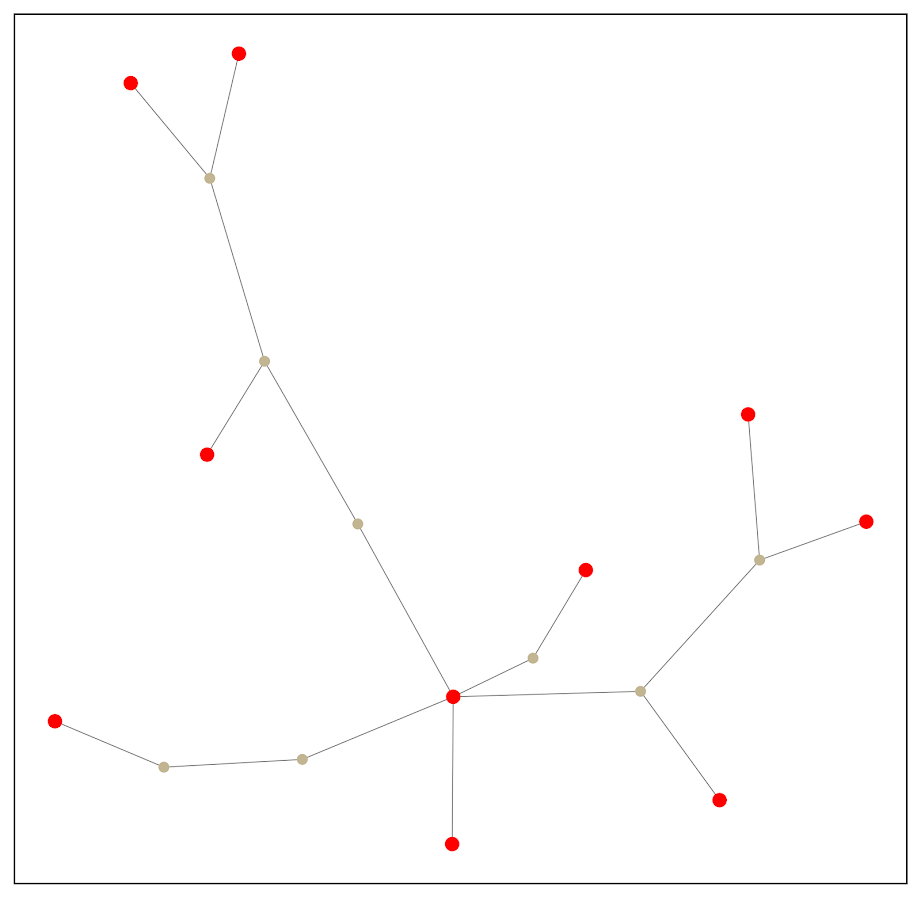Much simpler! And much more space to put additional labels.

I’ve posted the code and data to replicate here. Initially I debated on solving this via setting up the problem as a min-cost-flow, where one of the highlighted nodes had the supply, and the other highlighted nodes had the demand. But this approximate algorithm in my few tests looks really good in selecting tiny subsets, so not much need.

A few things to note about this. It is likely for many dense networks there will be many alternative subsets that are the same size, but different nodes (e.g. you can swap out a node and have the same looking network). A better approach to see connections between interesting nodes may be a betweenness centrality metric, where you only consider the flows between the highlighted nodes.

A partial solution to that problem is to add nodes/edges back in after the Steiner tree subset. Here is an example where I add back in all first degree nodes to the red nodes of interest: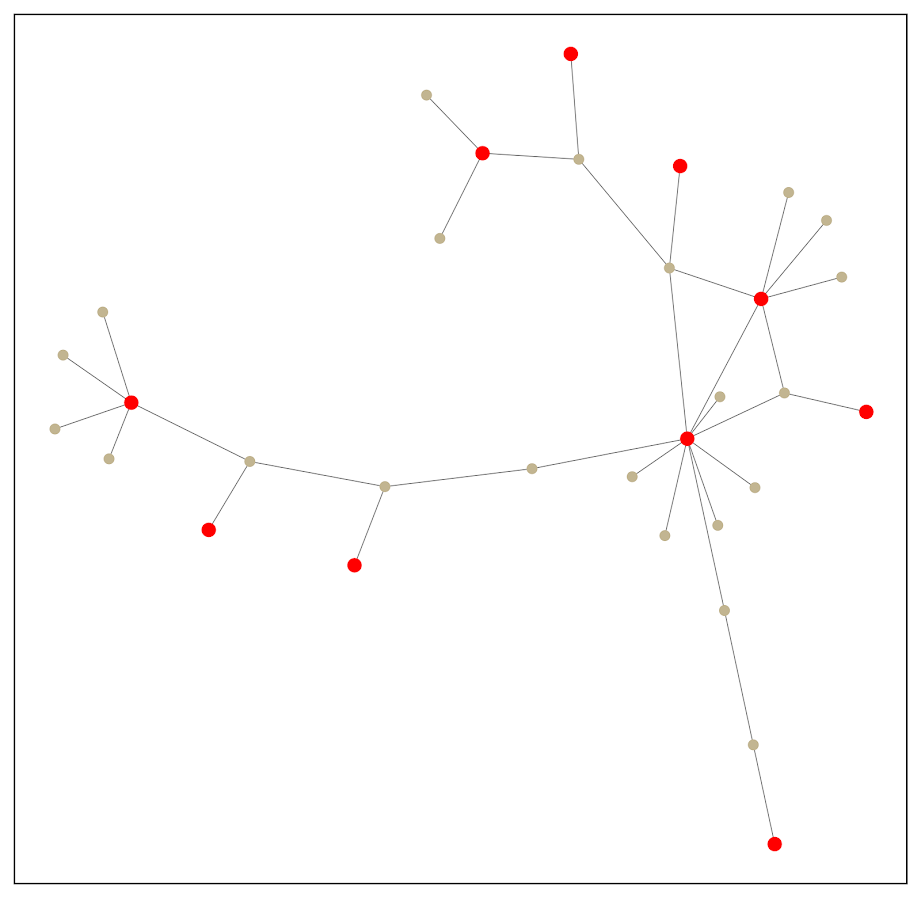So it is still a tiny enough network to plot. This just provides a way to identify higher order nodes of interest that aren’t directly connected to those red nodes.

# Histogram notes in python with pandas and matplotlib

Here are some notes (for myself!) about how to format histograms in python using pandas and matplotlib. The defaults are no doubt ugly, but here are some pointers to simple changes to formatting to make them more presentation ready.

First, here are the libraries I am going to be using.

import pandas as pd
import numpy as np
import matplotlib
from matplotlib import pyplot as plt
from matplotlib.ticker import StrMethodFormatter
from matplotlib.ticker import FuncFormatter

Then I create some fake log-normal data and three groups of unequal size.

#generate three groups of differing size
n = 50000
group = pd.Series(np.random.choice(3, n, p=[0.6, 0.35, 0.05]))
#generate log-normal data
vals = pd.Series(np.random.lognormal(mean=(group+2)*2,sigma=1))
dat = pd.concat([group,vals], axis=1)
dat.columns = ['group','vals']

And note I change my default plot style as well. So if you are following along your plots may look slightly different than mine.

One trick I like is using groupby and describe to do a simple textual summary of groups. But I also like transposing that summary to make it a bit nicer to print out in long format. (I use spyder more frequently than notebooks, so it often cuts off the output.) I also show setting the pandas options to a print format with no decimals.

#Using describe per group
pd.set_option('display.float_format', '{:,.0f}'.format)
print( dat.groupby('group')['vals'].describe().T )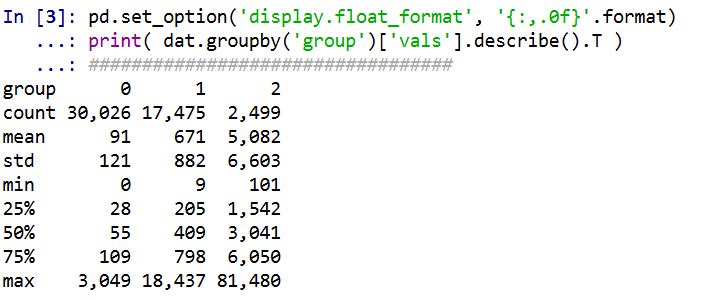Now onto histograms. Pandas has many convenience functions for plotting, and I typically do my histograms by simply upping the default number of bins.

dat['vals'].hist(bins=100, alpha=0.8)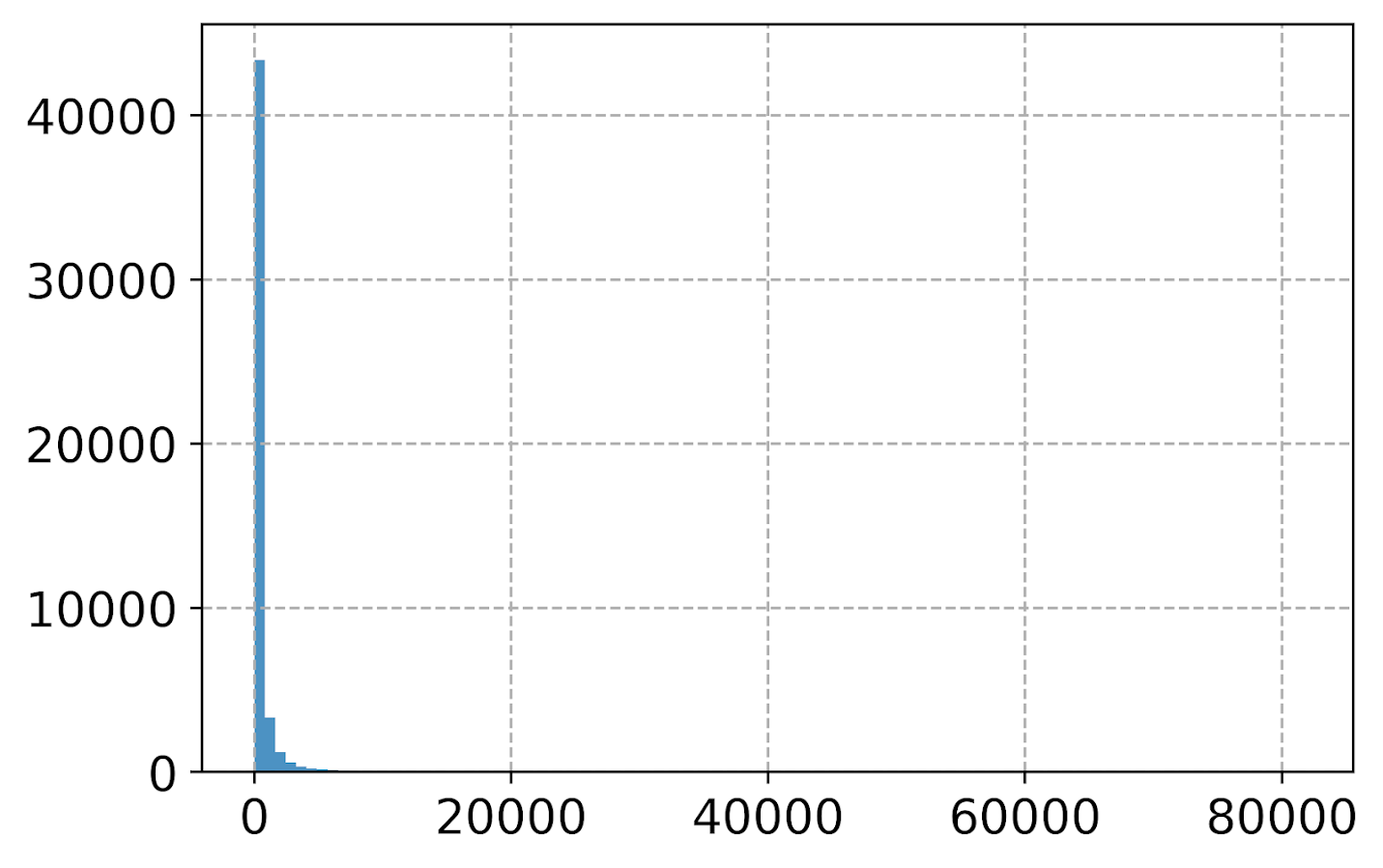Well that is not helpful! So typically when I see this I do a log transform. (Although note if you are working with low count data that can have zeroes, a square root transformation may make more sense. If you have only a handful of zeroes you may just want to do something like np.log([dat['x'].clip(1)) just to make a plot on the log scale, or some other negative value to make those zeroes stand out.)

#Histogram On the log scale
dat['log_vals'] = np.log(dat['vals'])
dat['log_vals'].hist(bins=100, alpha=0.8)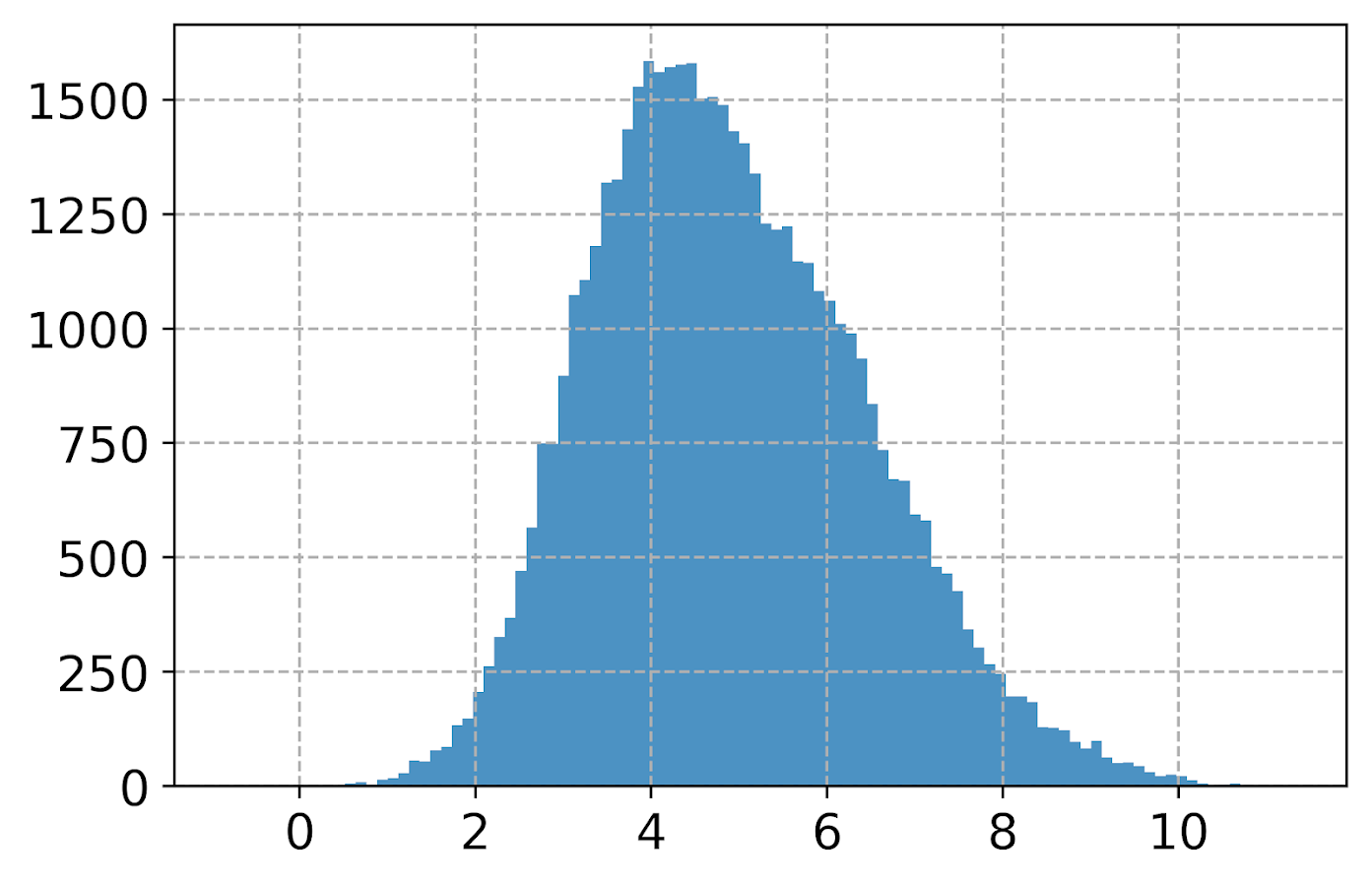Much better! It may not be obvious, but using pandas convenience plotting functions is very similar to just calling things like ax.plot or plt.scatter etc. So you can assign the plot to an axes object, and then do subsequent manipulations. (Don’t ask me when you should be putzing with axes objects vs plt objects, I’m just muddling my way through.)

So here is an example of adding in an X label and title.

#Can add in all the usual goodies
ax = dat['log_vals'].hist(bins=100, alpha=0.8)
plt.title('Histogram on Log Scale')
ax.set_xlabel('Logged Values')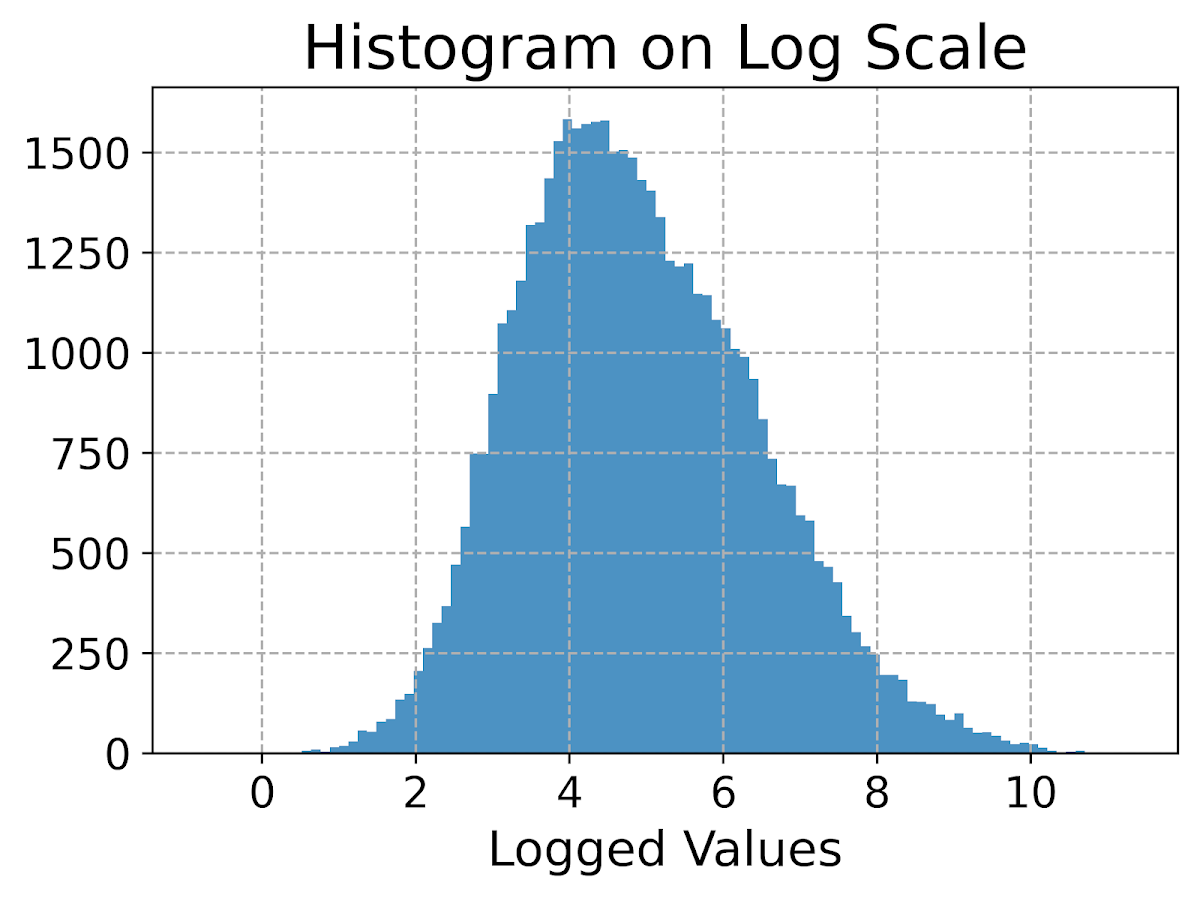Although it is hard to tell in this plot, the data are actually a mixture of three different log-normal distributions. One way to compare the distributions of different groups are by using groupby before the histogram call.

#Using groupby to superimpose histograms
dat.groupby('group')['log_vals'].hist(bins=100)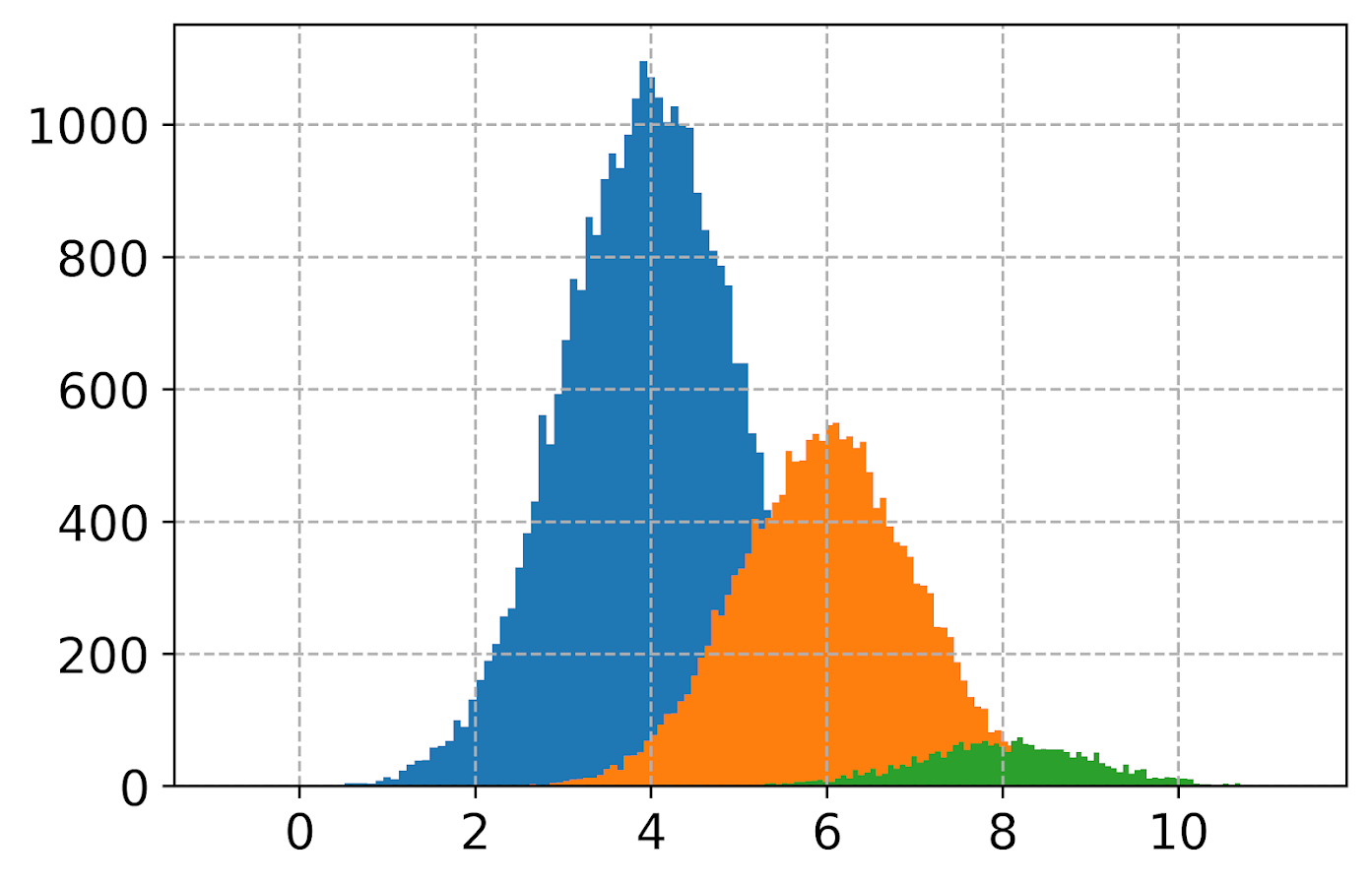But you see here two problems, since the groups are not near the same size, some are shrunk in the plot. The second is I don’t know which group is which. To normalize the areas for each subgroup, specifying the density option is one solution. Also plotting at a higher alpha level lets you see the overlaps a bit more clearly.

dat.groupby('group')['log_vals'].hist(bins=100, alpha=0.65, density=True)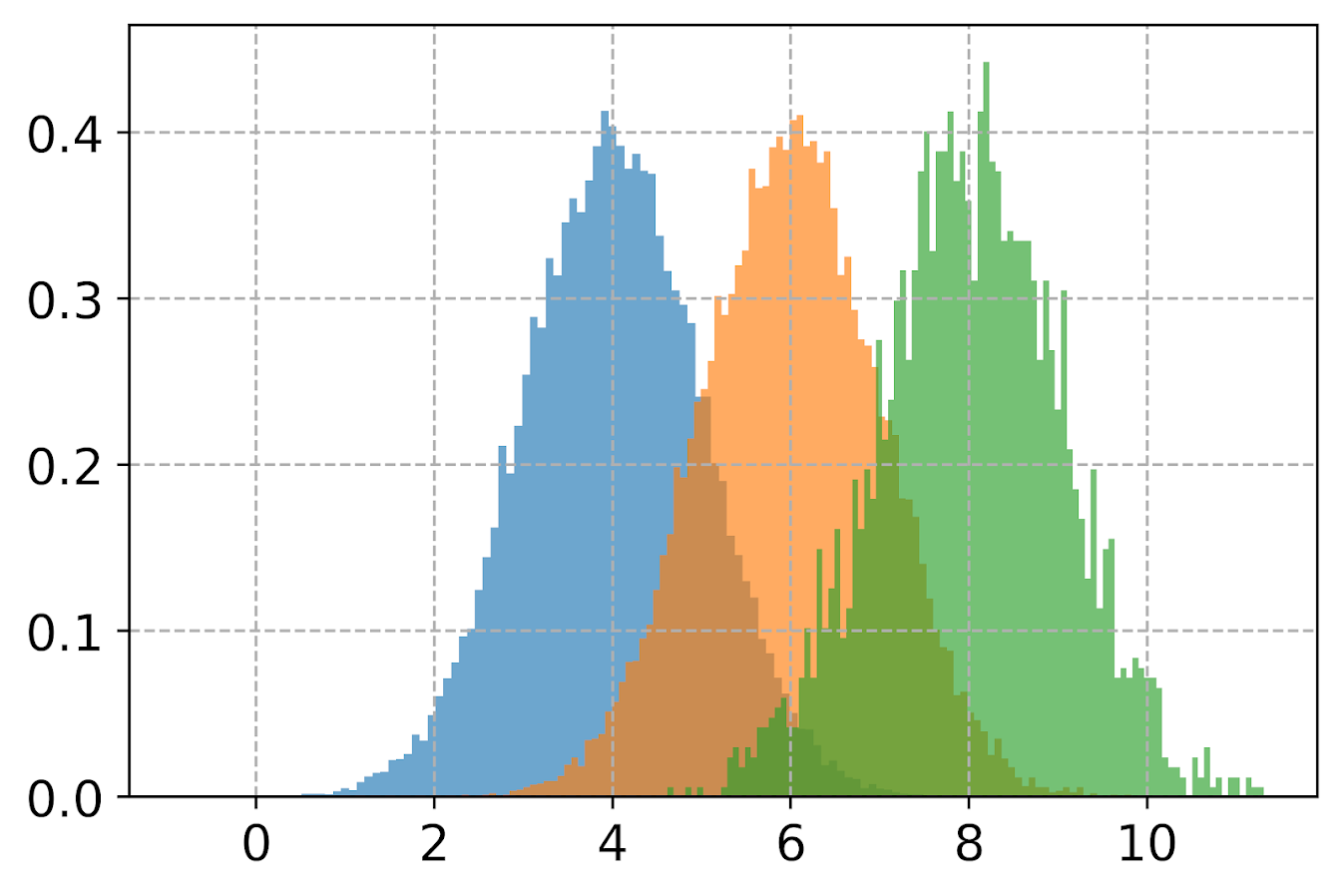Unfortunately I keep getting an error when I specify legend=True within the hist() function, and specifying plt.legend after the call just results in an empty legend. So another option is to do a small multiple plot, by specifying a by option within the hist function (instead of groupby).

#Small multiple plot
dat['log_vals'].hist(bins=100, by=dat['group'],
alpha=0.8, figsize=(8,8))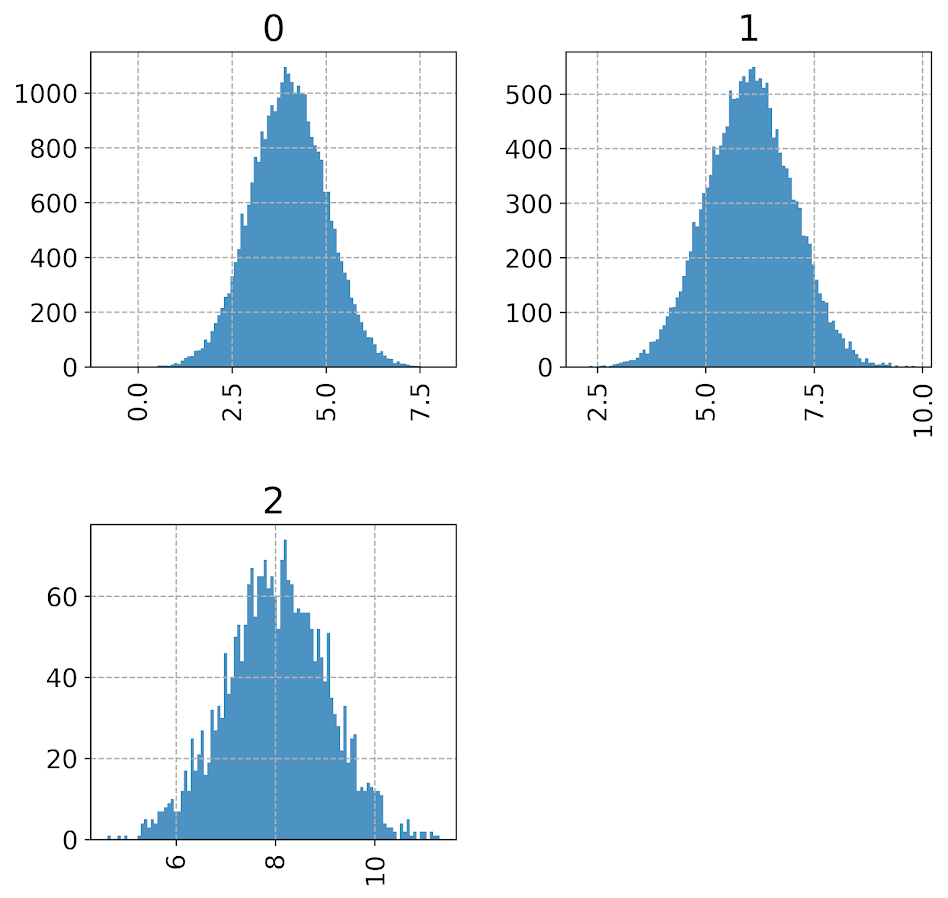This takes up more room, so can pass in the figsize() parameter directly to expand the area of the plot. Be careful when interpreting these, as all the axes are by default not shared, so both the Y and X axes are different, making it harder to compare offhand.

Going back to the superimposed histograms, to get the legend to work correctly this is the best solution I have come up with, just simply creating different charts in a loop based on the subset of data. (I think that is easier than building the legend yourself.)

#Getting the legend to work!
for g in pd.unique(dat['group']):
dat.loc[dat['group']==g,'log_vals'].hist(bins=100,alpha=0.65,
label=g,density=True)
plt.legend(loc='upper left')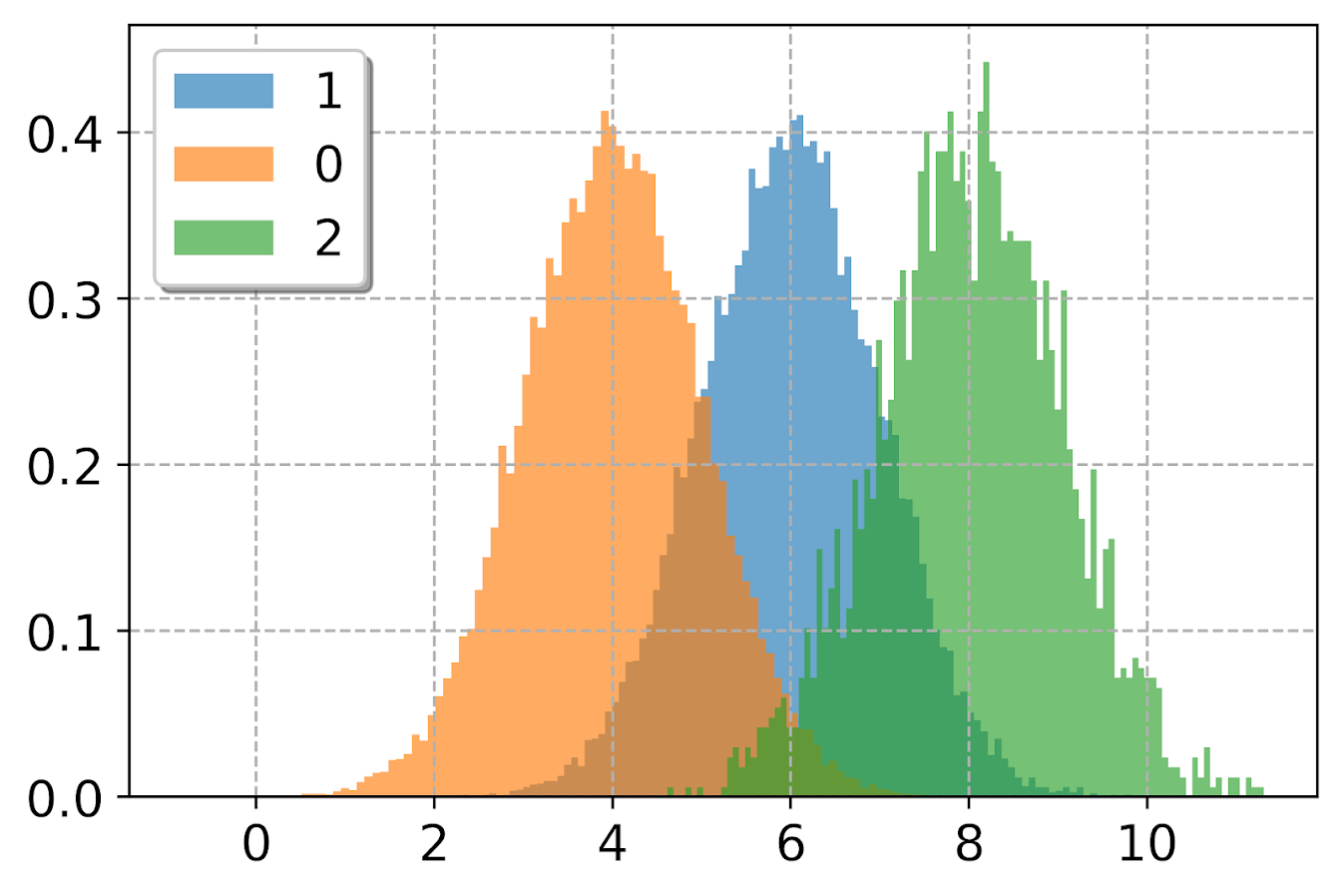Besides the density=True to get the areas to be the same size, another trick that can sometimes be helpful is to weight the statistics by the inverse of the group size. The Y axis is not really meaningful here, but this sometimes is useful for other chart stats as well.

#another trick, inverse weighting
dat['inv_weights'] = 1/dat.groupby('group')['vals'].transform('count')
for g in pd.unique(dat['group']):
sub_dat = dat[dat['group']==g]
sub_dat['log_vals'].hist(bins=100,alpha=0.65,
label=g,weights=sub_dat['inv_weights'])
plt.legend(loc='upper left')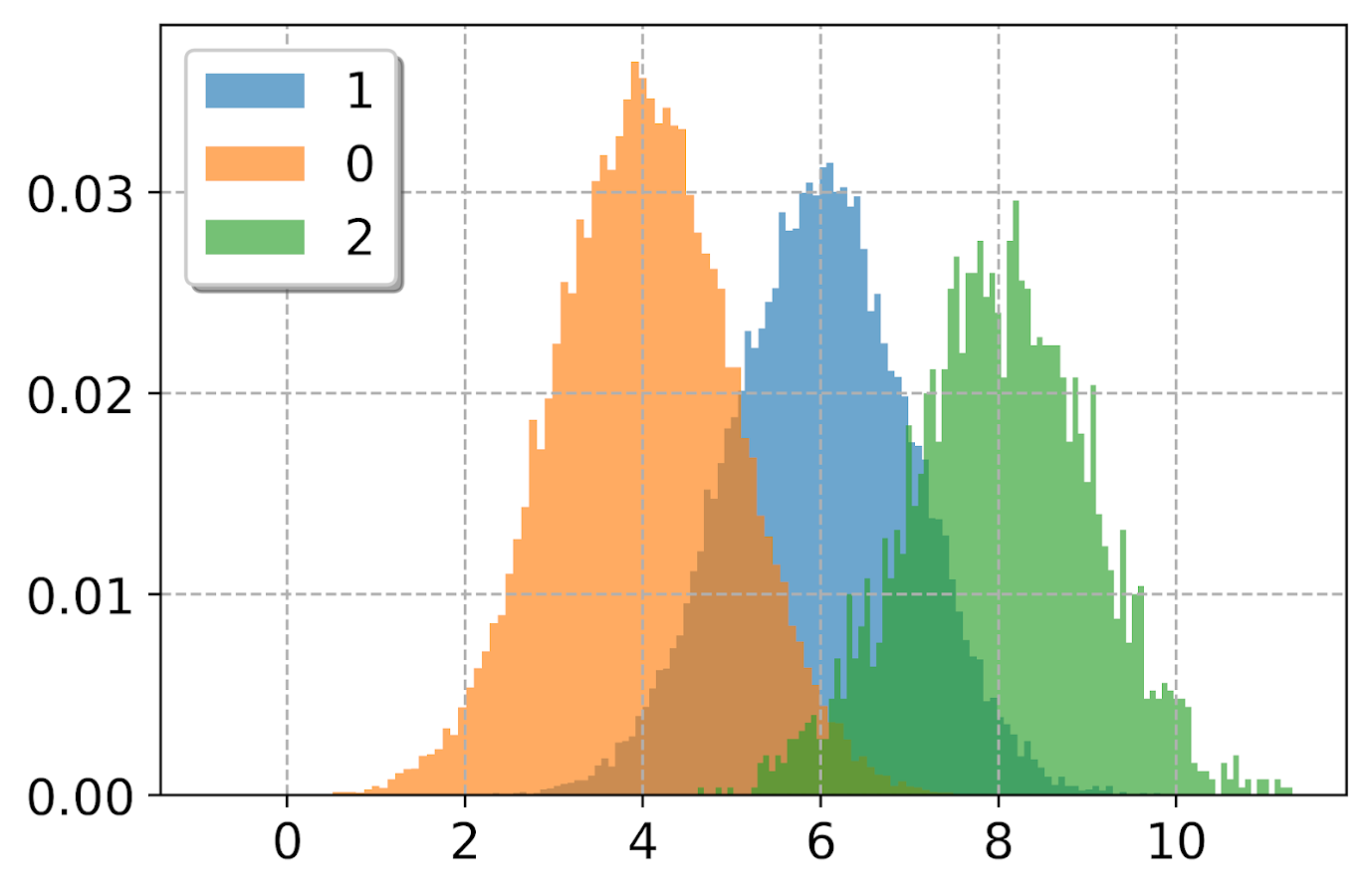So far, I have plotted the logged values. But I often want the labels to show the original values, not the logged ones. There are two different ways to deal with that. One is to plot the original values, but then use a log scale axis. When you do it this way, you want to specify your own bins for the histogram. Here I also show how you can use StrMethodFormatter to return a money value. Also rotate the labels so they do not collide.

#Specifying your own bins on original scale
#And using log formatting
log_bins = np.exp(np.arange(0,12.1,0.1))
ax = dat['vals'].hist(bins=log_bins, alpha=0.8)
plt.xscale('log', basex=10)
ax.xaxis.set_major_formatter(StrMethodFormatter('${x:,.0f}')) plt.xticks(rotation=45)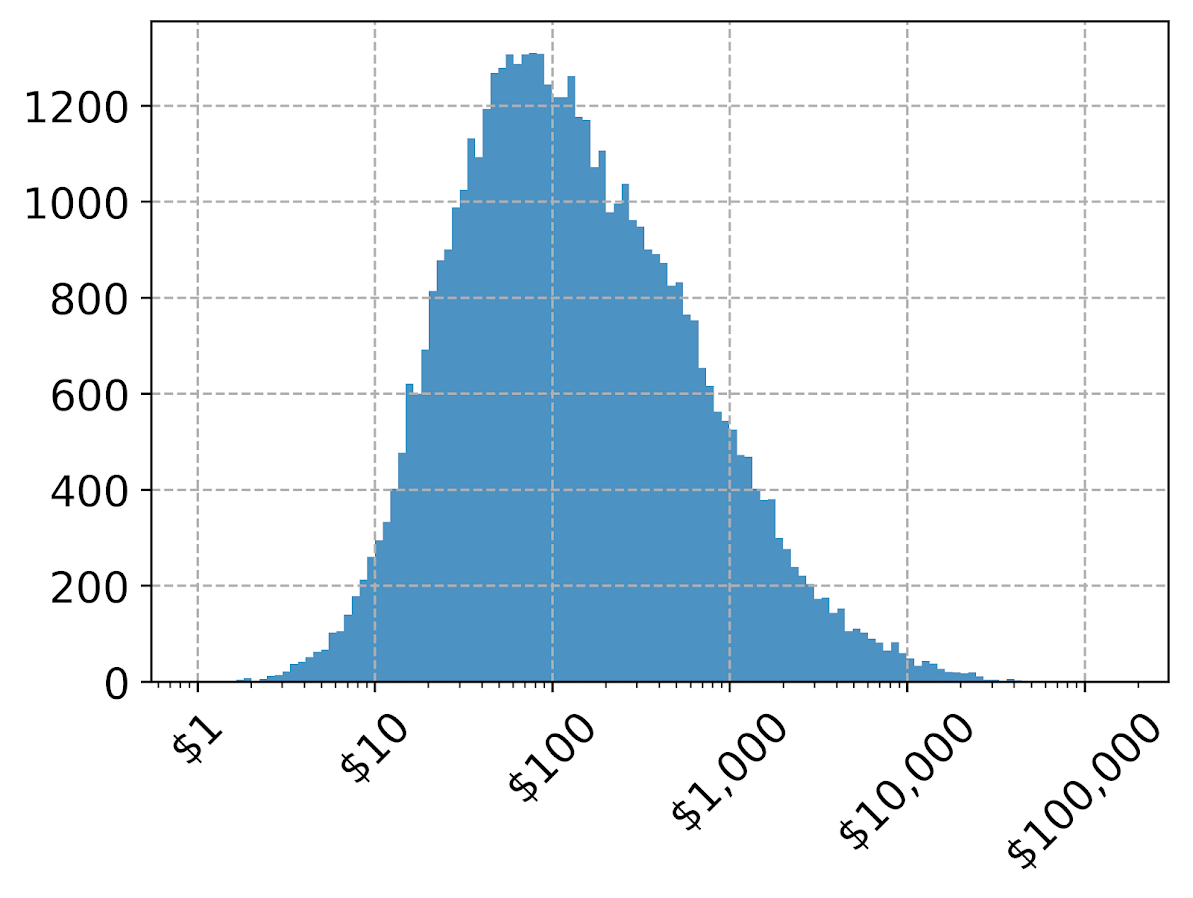If you omit the formatter option, you can see the returned values are 10^2, 10^3 etc. Besides log base 10, folks should often give log base 2 or log base 5 a shot for your data. Another way though is to use our original logged values, and change the format in the chart. Here we can do that using FuncFormatter. #Using the logged scaled, then a formatter #https://napsterinblue.github.io/notes/python/viz/tick_string_formatting/ def exp_fmt(x,pos): return '${:,.0f}'.format(np.exp(x))
fmtr = FuncFormatter(exp_fmt)

ax = dat['log_vals'].hist(bins=100, alpha=0.8)
plt.xticks(np.log([5**i for i in range(7)]))
ax.xaxis.set_major_formatter(fmtr)
plt.xticks(rotation=45)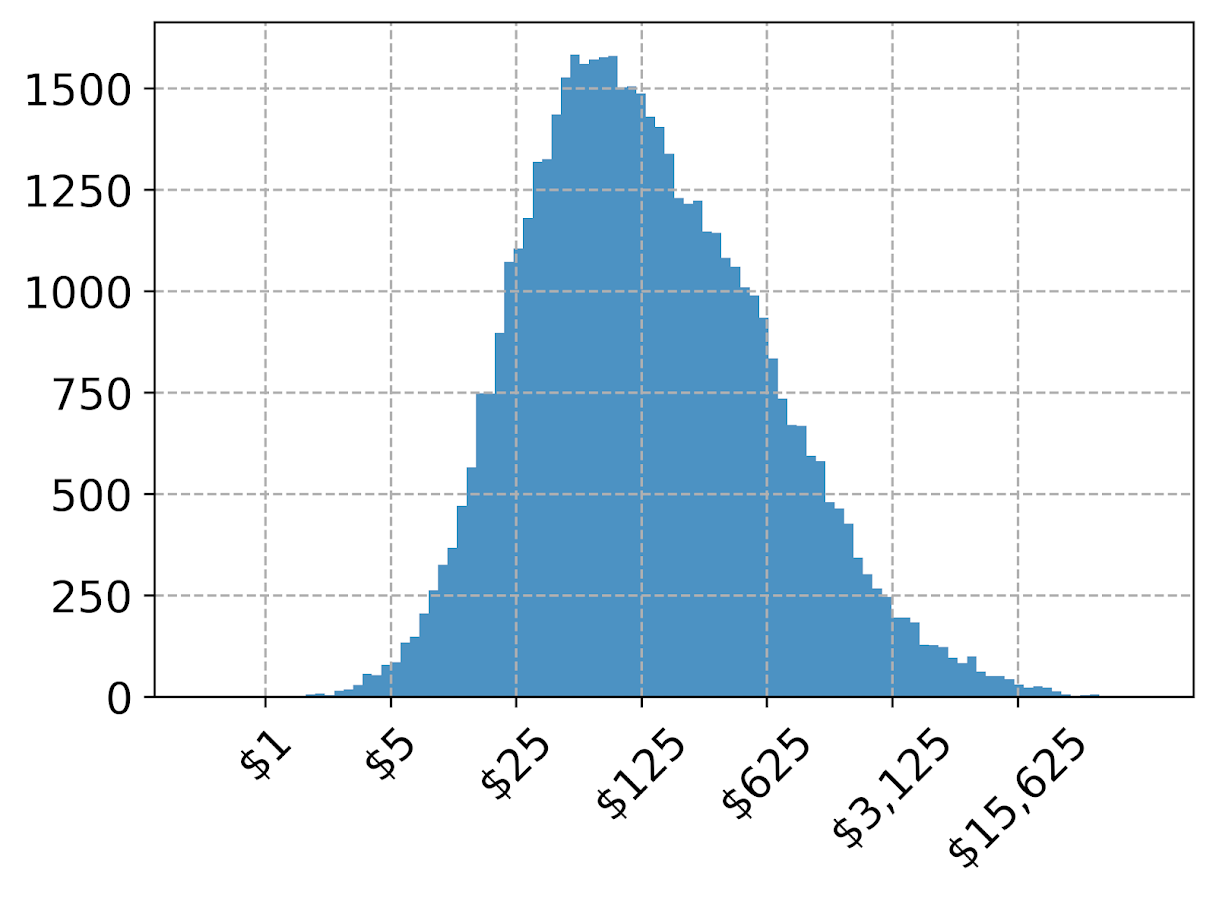On the slate is to do some other helpers for scatterplots and boxplots. The panda defaults are no doubt good for EDA, but need some TLC to make more presentation ready.

# An example of soft constraints in linear programming

Most of the prior examples of linear programming on my site use hard constraints. These are examples where I say to the model, “only give me results that strictly meet these criteria”, like “only select 40 cases to audit”, or “keep the finding rate over 50%”, etc.

There are alternative ways though to tell the model, “I want to select a finding rate over 50%, but still potentially consider those with lower finding rates”. One way to do that is via soft constraints, modifying the objective function directly to penalize (or favor) particular outcomes. For example, say you knew you could translate a 1% finding rate difference over 50% to a value of $1000. So if our original model is: Maximize Sum{D_i*Return_i} Subject To D_i element of (0,1) #decision variables are 0/1 Sum{D_i} = 100 #so select 100 cases We would then place an additional penalty term that looks like this: Maximize Sum{D_i*Return_i} + Sum{D_i*[(prob_i - 0.5)*1000]} Subject To D_i element of (0,1) Sum{D_i} = 100 So instead of a subject to constraint that says we need to be over 50% finding rate, we added a second penalty term for solutions that have an under 50% finding rate. So here if the finding rate in the end is 49%, it takes a hit of$1000 in the objective function. This example is also similar to a bi-objective function, here I just set an exact translation between finding rates and returns, but in practice often you don’t have that exact translation.

It just depends on your situation whether hard constraints or soft constraints make sense. Many situations you can swap one for the other, so different means same ends. For a good example of this, my allocating police resources paper on reducing disproportionate minority contact uses hard constraints (Wheeler, 2020), and George Mohler and colleagues have a very similar paper which uses soft constraints (Mohler et al., 2018). I imagine these will end up being very similar ends, although in that circumstance I prefer my hard constraint approach, as George’s you need to fiddle with the magnitude of the penalty term. Also I don’t tend to like changing the loss function for statistical/machine learning models, I just like changing what you do with the info after you have fit your model (Kleinberg et al., 2018).

Here I provide an example of where I think soft constraints make a bit of sense though. Imagine you have continuous predictive outputs, you need to make a binary yes/no decision among those options, but those predictive outputs also have a variance. An example of where this comes up is if you are making loan decisions, you want your portfolio to have a high return, but you also want to lower the variance of those returns as well.

For a simple example, imagine you are the lending institution and you have the choice between two scenarios:

• Scenario A: lend 1 person $100,000 with an expected return of$8,000, with a variance of $4,000 • Scenario B: lend 2 people$50,000, with an expected return of each for $4,000 each, with a variance of$1,000 for each loan

Since you expect to make the same amount of money under each scenario, option B is preferable if the loans are independent of each other (e.g. one going under does not cause the other to go under). In that case, variances are additive, so the total variance of option B is $2,000, so has much less volatility than does the A scenario. (Sorry to my criminology friends, this example is generic but I strain to find a criminal justice example to apply it to. It would not be crazy that you have low volatility vs high volatility hot spots. So you may want to choose a consistent hot spot as oppossed to a fleeting one for an intervention. But I don’t think that will happen in practice quite like that. Choosing among expensive high risk/reward vs inexpensive treatment regimes low risk/reward in corrections settings may also make sense, but that is crazy pie in the sky technocratic given the current state of affairs as well.) # Example with Lending Club Data So to illustrate an example with actual data, I’ve provide Python code fitting a predictive model to Lending Club data on loans. (I got the original dataset from Kaggle.) I am just going to highlight some key points here in the blog post. You will need to go to the code to see everything. First, I’ve been introduced to this dataset as predicting a binary default/no-default. I have code doing that in the code snippet as well, and it performed OK. But it was very uncalibrated as to whether my portfolio made money – so even though the default estimates were pretty well spot on, my portfolios did not make much money. This is because people who default pay back some loans, and also quite a few people in the dataset pay back the loans fast, so the lenders don’t make as much as interest as you would expect at the start of the loan. So I cut out the middle man and just estimated a random forest model predicting the actual money one made on the loan. I only kept cases that are either 'Fully Paid','Charged Off', 'Default', so I don’t model loans that are still ongoing. I end up modeling then the value total_pymnt - loan_amnt. You can look into the code to see the variables I included in the model, but one of the neat things about regression random forests is that you can not only get the mean prediction, you can also look at the variance over all the trees. See below a function to do that (in the 01 py file): ############################################################### #Fit random forest model model = RandomForestRegressor(n_estimators=1000, random_state=10, min_samples_leaf=100) model.fit(train[x_vars], train[y_var]) #Check the predicted vs actual on the test set y_pred = model.predict(test[x_vars]) #predicted mean test['y_pred'] = y_pred #I want an estimate of the variance def tree_var(X, rf_mod): per_tree_pred = [pd.Series(tree.predict(X), index=X.index) for tree in rf_mod.estimators_] pd_res = pd.concat(per_tree_pred, axis=1) pd_var = pd_res.var(axis=1) return pd_var test['y_var'] = tree_var(X=test[x_vars], rf_mod=model) ############################################################### And that predicted value and variance are then what I feed into my subsequent linear programming problem (in the 02 py file). The model in some more text is: Maximize Sum{D_i*(prediction - lambda*variance)} Subject To: D element of (0,1) #decision variables Sum{D_i*loan_amount_i} <= 300000 #only have so much$ to loan, so no leveraging


Where lambda is the tuner – higher variances will get higher penalties. So going back to our two loan example, if lambda = 1, scenario A it would be 8000 - 1*4000 = 4000, and scenario B would be 8000 - 1*2000 = 6000, so that penalty would choose scenario B over A. Whereas without the penalty the two scenarios are exactly the same.

Since the lambda value is arbitrary, I illustrate the approach selecting portfolios of loans that are a total of $300,000 (I divided the loans by$1000 to make the numbers a bit easier to view). This is a totally held out sample of around 5k loans. So you can see my first model (with no constraints):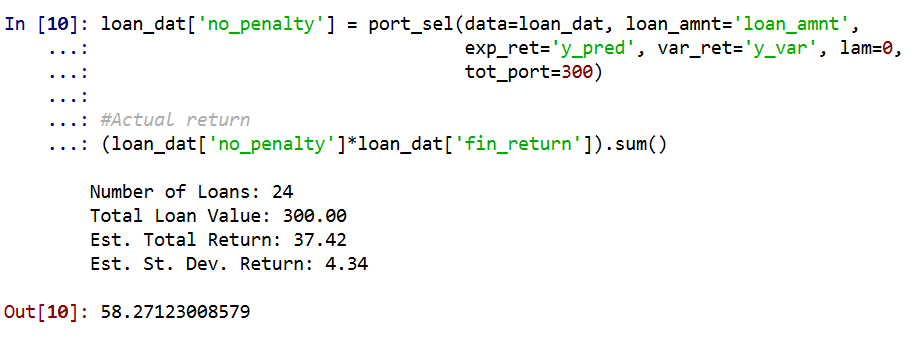And my second model with a higher lambda value of 1 selects more (smaller) loans, and reduces the variance. You can see since we have the actual outcomes, I can show that both portfolios turned a profit, each above what I predicted. But the standard deviation for second portfolio is cut not quite in half.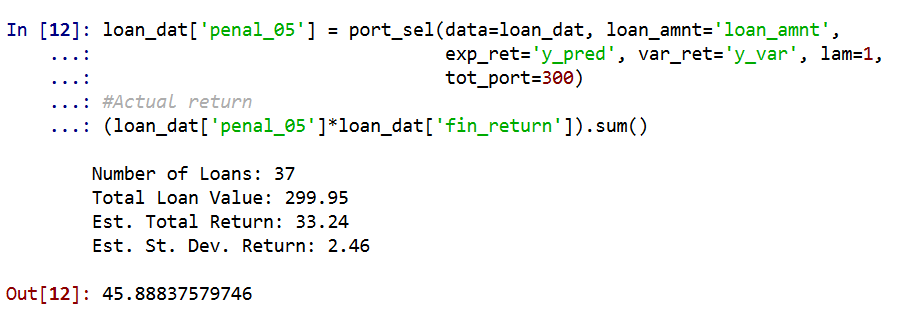So you can see in that one selection it worked out OK, but this does not verify that my variance estimates are correct (they are no doubt too small, as you can see the actual returns are way higher than I predicted).

To test them out though I do a simulation. I draw 1000 cases out of those 5000, and then again pick $300k in loans. I do that process 1000 times under a set of different lambda penalty terms, [0, 0.5, 1.0, 2.0, 3.0]. For those simulations, here is the overall distribution of the returns under different penalty terms for the variance.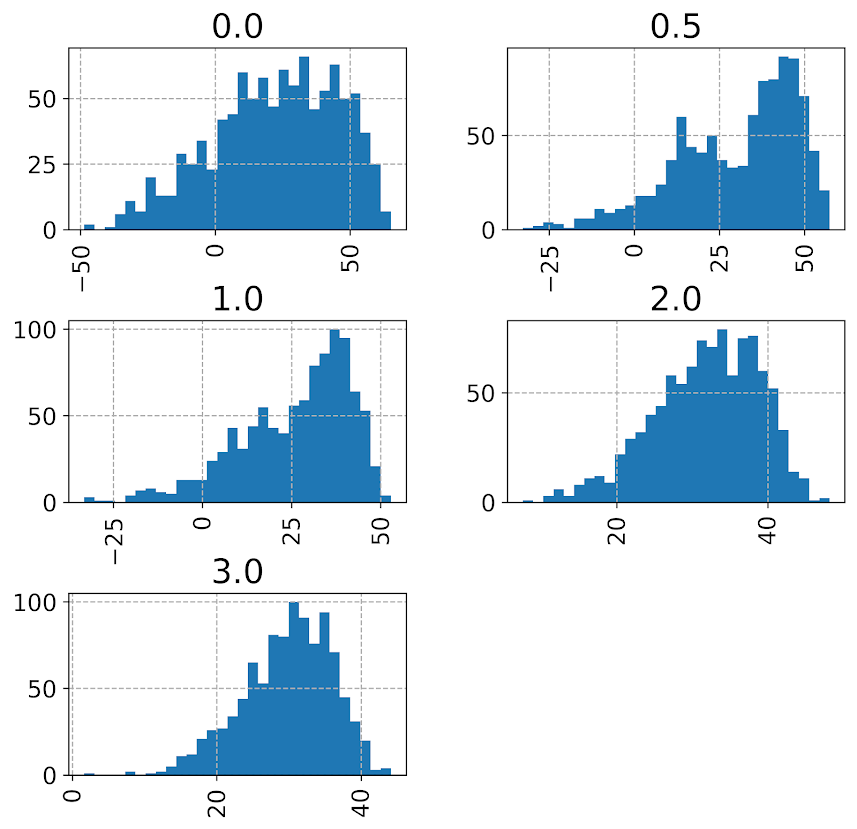Note that those histograms have different X axes. It is easier to see the moments of the spread in a boxplot: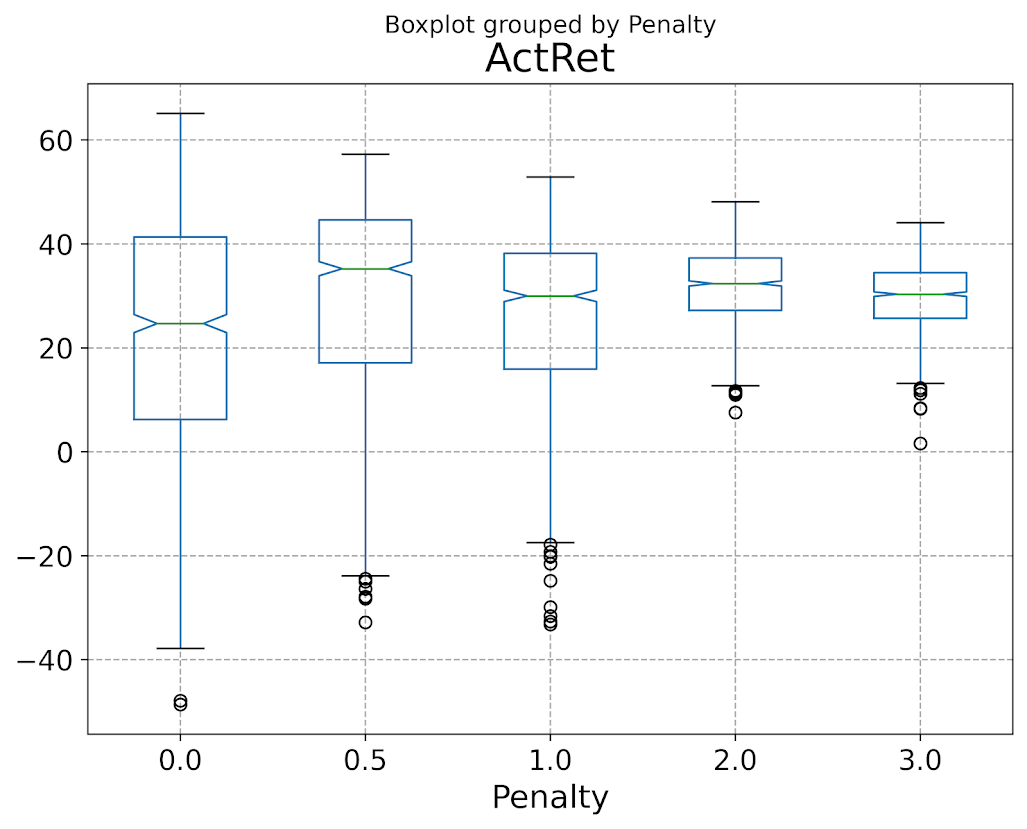So here you can see each scenario has pretty near the same median return (somewhere around$30k), but the penalties reduce the variance. The higher penalties end up selecting portfolio’s that always at least make money, whereas the lower penalty terms you do end up losing money in some scenarios.

Unfortunately I did not beat the market in my simple weekend experimentation, so don’t sink a bunch of money into Lending club based on this! The average returns starting from \$300k are something like an annualized rate of return of around 3% (over 3 years) for the smaller simulation pick from 1000. These include loans of 60 months as well though. So even though my linear program with the penalty term did a good job of reducing the risk, this isn’t good enough returns for me to put a bunch of my money into Lending Club.

But there is no doubt improvements both to the modeling as well as the portfolio selection. For modeling I would be tempted to try out a discrete time survival model for payments over time, but that would be more work than I could do in a weekend. (Also I only incorporated the easy continuous variables here I could prepare in just a few minutes, so maybe more feature engineering would boost my results.)

I could also adapt the linear program to take into account covariance between the loans, but not sure how to estimate them (a multi-level model perhaps?). You also may want to do some sort of conditional value at risk approach in the linear program, say instead of piping in the variance from random forests, count up how often you lose money and put that as a penalty or constraint on the system.